# JP4388965B2 - Clock gating analysis program, recording medium recording the program, clock gating analysis device, and clock gating analysis method - Google Patents

Clock gating analysis program, recording medium recording the program, clock gating analysis device, and clock gating analysis method Download PDF

## Info

Publication number
JP4388965B2
JP4388965B2 JP2007032540A JP2007032540A JP4388965B2 JP 4388965 B2 JP4388965 B2 JP 4388965B2 JP 2007032540 A JP2007032540 A JP 2007032540A JP 2007032540 A JP2007032540 A JP 2007032540A JP 4388965 B2 JP4388965 B2 JP 4388965B2
Authority
JP
Japan
Prior art keywords
state
clock gating
state transition
output
clock
Prior art date
Legal status (The legal status is an assumption and is not a legal conclusion. Google has not performed a legal analysis and makes no representation as to the accuracy of the status listed.)
Expired - Fee Related
Application number
JP2007032540A
Other languages
Japanese (ja)
Other versions
JP2008197920A (en
Inventor

Original Assignee

Priority date (The priority date is an assumption and is not a legal conclusion. Google has not performed a legal analysis and makes no representation as to the accuracy of the date listed.)
Filing date
Publication date
Application filed by 富士通株式会社 filed Critical 富士通株式会社
Priority to JP2007032540A priority Critical patent/JP4388965B2/en
Publication of JP2008197920A publication Critical patent/JP2008197920A/en
Application granted granted Critical
Publication of JP4388965B2 publication Critical patent/JP4388965B2/en
Expired - Fee Related legal-status Critical Current
Anticipated expiration legal-status Critical

• 244000241601 filaree Species 0.000 title claims description 727
• 238000004458 analytical methods Methods 0.000 title claims description 194
• 238000006243 chemical reactions Methods 0.000 claims description 99
• 238000000034 methods Methods 0.000 claims description 93
• 238000004088 simulation Methods 0.000 claims description 52
• 230000000875 corresponding Effects 0.000 claims description 30
• 238000004364 calculation methods Methods 0.000 claims description 29
• 238000000605 extraction Methods 0.000 claims description 25
• 230000004913 activation Effects 0.000 claims description 22
• 239000000203 mixtures Substances 0.000 description 81
• 238000010586 diagrams Methods 0.000 description 33
• 230000000576 supplementary Effects 0.000 description 30
• 239000000284 extracts Substances 0.000 description 7
• 238000004519 manufacturing process Methods 0.000 description 4
• 230000003287 optical Effects 0.000 description 3
• 280000115721 A Line companies 0.000 description 2
• 230000005540 biological transmission Effects 0.000 description 2
• 230000000694 effects Effects 0.000 description 2
• 230000012447 hatching Effects 0.000 description 2
• 280000753989 Data System companies 0.000 description 1
• 230000015572 biosynthetic process Effects 0.000 description 1
• 238000005516 engineering processes Methods 0.000 description 1
• 230000002779 inactivation Effects 0.000 description 1
• 239000004065 semiconductors Substances 0.000 description 1
• 238000003786 synthesis reactions Methods 0.000 description 1
• 230000002194 synthesizing Effects 0.000 description 1

## Images

•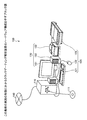•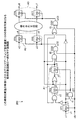••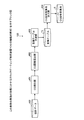•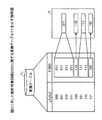•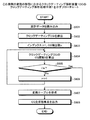•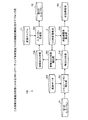•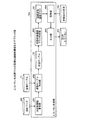•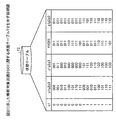••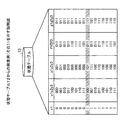•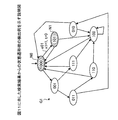•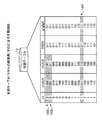••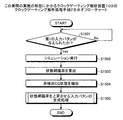•••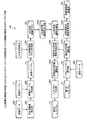•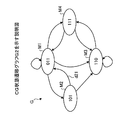•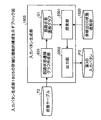•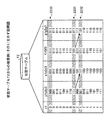•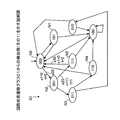•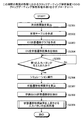•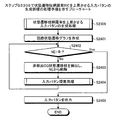•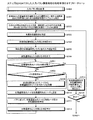•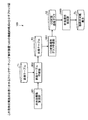•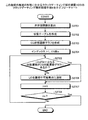•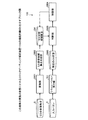••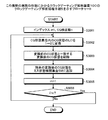•## Classifications

• GPHYSICS
• G06COMPUTING; CALCULATING; COUNTING
• G06FELECTRIC DIGITAL DATA PROCESSING
• G06F30/30Circuit design
• G06F30/32Circuit design at the digital level
• G06F30/33Design verification, e.g. functional simulation or model checking
• G06F30/3308Design verification, e.g. functional simulation or model checking using simulation
• G06F30/3312Timing analysis

## Description

The present invention relates to a clock gating analysis program for analyzing clock gating incorporated in a semiconductor integrated circuit (LSI), a recording medium recording the program, a clock gating analysis device, and a clock gating analysis method. Clock gating (function) is one of the main technologies for reducing the power consumption of LSIs. The clock signals that are not calculated in the LSI are stopped at the root of clock signal propagation as much as possible, and unnecessary clocks are used. This technology reduces power consumption due to signal propagation.

Conventionally, clock gating has been manually inserted in units of large blocks, so that the designer can fully understand the operation of the clock gating function. Therefore, in the verification of clock gating so far, (1) timing verification of a clock signal for verifying whether the timing at which each clock gating control signal arrives is within a normal operation range (for example, Patent Document 1 below) , 2) and (2) timing verification of data system signals in consideration of the clock gating function (for example, see Non-Patent Document 1).

Further, as verification other than clock gating timing verification, a tool that extracts and draws a partial circuit related to clock gating has been disclosed (for example, see Patent Document 3 below).

Japanese Unexamined Patent Publication No. Hei 8-20269 JP 11-259554 A Japanese Patent Laid-Open No. 10-283381 M. Kawarabayashi et al .: "A Verification Technique for Gated Clock", Proceedings of the Design Automation Conference, pp.123-127, 1993

However, there is an increasing demand for lower power consumption of LSIs, and clock gating is inserted more finely or automatically. The number of clock gatings inserted has increased significantly, making it almost impossible for designers to understand the relationship between individual clock gatings in an integrated manner.

In such a situation, the omission of verification of the clock gating function itself has become a problem. Specifically, if the clock is not stopped where it should be stopped, or if it is stopped where the clock should not be stopped, it will appear as a functional bug, so a design failure due to verification failure is a problem. In addition, if it is better to stop the clock, but it is not stopped, there is a problem that it is not a function bug, but wastes power. In recent years, the power consumption has been severely limited, and even a slight increase often leads to over-target power, which often causes problems.

The present invention eliminates the problems caused by the prior art described above, and therefore, a clock gating analysis program capable of realizing a reduction in design burden on a designer, improvement in reliability of a circuit to be analyzed, and reduction in power consumption, It is an object of the present invention to provide a recording medium on which the program is recorded, a clock gating analysis apparatus, and a clock gating analysis method.

In order to solve the above-described problems and achieve the object, a clock gating analysis program, a recording medium recording the program, a clock gating analysis device, and a clock gating analysis method according to the present invention are provided in an analysis target circuit. A clock signal for supplying a clock to the sequential circuit element or stopping the clock is detected, and a control signal input from the sequential circuit element to an input terminal other than the clock input terminal in the detected clock gating The clock gating function expressing activation / deactivation of the local clock output from the clock gating is calculated for each clock gating, and the calculated clock gating function Substituting a combination of output values from circuit elements , By calculating the combination of the values of the clock gating functions as a clock gating state representing the mutual activation / deactivation of the clock gating from the combination of output values from the sequential circuit elements. A conversion table representing conversion to a clock gating state is created, and based on the created conversion table, a clock gating all-state set covering all the clock gating states in the analysis target circuit is output. And

According to the present invention, in the clock gating analysis, all clock gating states introduced in order to take into account the state change between individual clock gatings can be automatically covered.

Further, in the above invention, the clock gating state detected by a simulation result obtained by giving an arbitrary input pattern to the analysis target circuit covers the clock gating whole state set output by the all state set output step. It is good also as calculating the state coverage rate showing the ratio which is present.

According to the present invention, it is possible to check how many omissions of verification of a clock gating bug that causes a functional bug of a circuit to be analyzed by simulation exist.

In the above invention, a clock gating state (hereinafter, referred to as a “detected clock gating state”) excluding a clock gating state (hereinafter referred to as “detected clock gating state”) detected by a simulation result in which an arbitrary input pattern is given to the analysis target circuit. (Referred to as “non-detected clock gating state”) is extracted from the clock gating total state set, and an input pattern for causing the extracted non-clock gating state to appear is generated using the conversion table. Good.

According to the present invention, it is possible to automatically generate an input pattern for causing clock gating not to be covered by the clock gating total state set. Thereby, the state coverage rate can be increased.

Further, in the above invention, a next state function representing an output change from the current time to the next time with respect to each sequential circuit element is calculated, and each sequential circuit element is based on the conversion table and the calculated next state function. A state in which a combination of output values at the present time from the clock and a clock gating state according to the combination are associated with a combination of output values at the next time from the sequential circuit elements and a clock gating state according to the combination Creating a table, creating a circuit state transition graph representing a state transition of the analysis target circuit based on a combination of output values of the current time and the next time from each sequential circuit element in the created state table, Created by setting the non-detected clock gating state to the clock gating state of the next time. In the circuit state transition graph, the state transition from the combination of the output value of the next time corresponding to the non-detection clock gating state to the initial state is searched, and the searched search result is obtained as the extracted non-clock gating state. It is good also as outputting as an input pattern made to appear.

According to the present invention, it is possible to automatically generate an input pattern for causing clock gating not to be covered by the clock gating total state set. Thereby, the state coverage rate can be increased.

Further, in the above invention, a next state function representing an output change from the current time to the next time with respect to each sequential circuit element is calculated, and each sequential circuit element is based on the conversion table and the calculated next state function. A state in which a combination of output values at the present time from the clock and a clock gating state according to the combination are associated with a combination of output values at the next time from the sequential circuit elements and a clock gating state according to the combination A clock gating state transition graph representing the state transition of the clock gating state is created based on the generated clock gating total state set and the created state table, and the generated clock gate State transition of the clock gating state from the gating state transition graph The by extracting all, may output the clock gating all state transition branch group.

According to the present invention, in the clock gating analysis, all clock gating state transition branches introduced to take into account the state change between individual clock gatings can be automatically covered.

In the above invention, the ratio of the clock gating state transition branches detected from the simulation result obtained by applying an arbitrary input pattern to the analysis target circuit covers the output clock gating all state transition branch set. It is also possible to calculate the state transition branch coverage ratio.

According to the present invention, it is possible to check how many omissions of verification of a clock gating bug that causes a functional bug of a circuit to be analyzed by simulation exist.

Also, in the above invention, clock gating excluding a clock gating state transition branch (hereinafter referred to as “detected clock gating state transition branch”) detected by a simulation result in which an arbitrary input pattern is given to the analysis target circuit. A state transition branch (hereinafter referred to as a “non-detected clock gating state transition branch”) is extracted from the set of all clock gating state transition branches, and the extracted non-clock gating state transition is extracted using the conversion table. It is good also as producing | generating the input pattern which makes a branch appear.

According to the present invention, it is possible to automatically generate an input pattern for causing clock gating that is not covered by the clock gating all state transition branch set. This also increases the state transition branch coverage rate.

Further, in the above invention, a circuit state transition graph representing a state transition of the analysis target circuit is created based on a combination of output values of the current time and the next time from each sequential circuit element in the state table, By making the detected clock gating state transition branch a clock gating state transition branch at the next time, in the created circuit state transition graph, from the circuit state transition branch corresponding to the non-clock gating state transition branch to the initial state The state transition may be searched, and the searched search result may be output as an input pattern for causing the non-clock gating state transition branch to appear.

According to the present invention, it is possible to automatically generate an input pattern for causing clock gating that is not covered by the clock gating all state transition branch set. This also increases the state transition branch coverage rate.

Further, in the above invention, a next state function representing an output change from the current time to the next time with respect to each sequential circuit element is calculated, and each sequential circuit element is based on the conversion table and the calculated next state function. A state in which a combination of output values at the present time from the clock and a clock gating state according to the combination are associated with a combination of output values at the next time from the sequential circuit elements and a clock gating state according to the combination A clock gating state transition graph representing the state transition of the clock gating state is created based on the generated clock gating total state set and the created state table, and the generated clock gate Clock gating is deactivated from the gating state transition graph May be extracted state transitions not return to Luo activated state.

According to the present invention, bug detection of the clock gating function can be comprehensively performed.

In the above invention, any clock gating state in the output clock gating total state set is converted into a clock gating state in which any local clock is changed from the activated state to the deactivated state, and converted. A clock gating miss state candidate may be generated by deleting a clock gating state that matches the clock gating state in the clock gating total state set from the later clock gating states.

According to the present invention, it is not necessary to activate the clock (the state in which the clock is supplied), but the clock gating miss state candidate in the activated state (the state in which the clock is stopped) is intentionally Can be automatically generated.

Further, in the above invention, it is determined whether the generated clock gating error state candidate includes a specific clock gating state detected by a simulation result in which an arbitrary input pattern is given to the analysis target circuit. And determining whether or not the output value of the sequential circuit element driven by the clock gating to be converted changes, and based on the determined determination result, from the clock gating error state candidate to the specific The clock gating state may be deleted.

According to the present invention, clock gating miss state candidates can be narrowed down based on the simulation result.

According to the clock gating analysis program, the recording medium on which the program is recorded, the clock gating analysis device, and the clock gating analysis method according to the present invention, the design burden on the designer is reduced and the reliability of the analysis target circuit is improved. And, there is an effect that low power consumption can be realized.

Exemplary embodiments of a clock gating analysis program, a recording medium recording the program, a clock gating analysis apparatus, and a clock gating analysis method according to the present invention will be explained below in detail with reference to the accompanying drawings. .

(Hardware configuration of clock gating analyzer)
First, the hardware configuration of the clock gating analyzer according to the embodiment of the present invention will be described. FIG. 1 is a block diagram showing a hardware configuration of a clock gating analysis apparatus according to an embodiment of the present invention.

In FIG. 1, a clock gating analysis device 100 is composed of a computer main body 110, an input device 120, and an output device 130. A network such as a LAN, WAN, or the Internet via a router or a modem (not shown). 140 can be connected.

The computer main body 110 has a CPU, a memory, and an interface. The CPU controls the entire clock gating analysis apparatus 100. The memory is composed of ROM, RAM, HD, optical disk 111, and flash memory. The memory is used as a work area for the CPU.

Various programs are stored in the memory, and loaded according to instructions from the CPU. Data read / write of the HD and the optical disk 111 is controlled by a disk drive. The optical disk 111 and the flash memory are detachable from the computer main body 110. The interface controls input from the input device 120, output to the output device 130, and transmission / reception with respect to the network 140.

The input device 120 includes a keyboard 121, a mouse 122, a scanner 123, and the like. The keyboard 121 includes keys for inputting characters, numbers, various instructions, and the like, and inputs data. Further, it may be a touch panel type. The mouse 122 performs cursor movement, range selection, window movement, size change, and the like. The scanner 123 optically reads an image. The read image is captured as image data and stored in a memory in the computer main body 110. Note that the scanner 123 may have an OCR function.

The output device 130 includes a display 131, a printer 132, a speaker 133, and the like. The display 131 displays data such as a document, an image, and function information as well as a cursor, an icon, or a tool box. The printer 132 prints image data and document data. The speaker 133 outputs sound such as sound effects and reading sounds.

(Example of analysis target circuit)
Next, an analysis target circuit to be analyzed by the clock gating analysis apparatus 100 according to the embodiment of the present invention will be described. FIG. 2 is a circuit diagram showing an example of an analysis target circuit to be analyzed by the clock gating analysis apparatus 100 according to the embodiment of the present invention.

In FIG. 2, the analysis target circuit 200 is expressed by design data such as a net list after logic synthesis. The analysis target circuit 200 is provided with clock gating CGi (i = 1, 2,...). The clock gating CGi is a circuit that controls the output of a clock output to a sequential circuit element (for example, a flip-flop circuit; hereinafter referred to as “FF”), and is, for example, a logic gate such as an AND circuit. It is configured.

More specifically, the output terminal of the clock gating CGi is connected to the clock terminal of the FF. One input terminal captures the clock, and the other input terminal captures the control signal, so that the clock gating is performed. Or output to the clock terminal of the sequential circuit element of the output destination (directly or indirectly). As described above, the clock clk output from the clock gating CGi to the sequential circuit element that is the output destination is referred to as a local clock LCi.

An FF that receives the same local clock LCi from the clock terminal is referred to as “FF group Ai of local clock LCi”. For example, the FF group A1 of the local clock LC1 includes FF2 and FF3, the FF group A2 of the local clock LC2 includes FF4 and FF5, and the FF group A3 of the local clock LC3 includes FF6 and FF7.

Since FF1 does not capture any local clock LCi, it does not belong to any FF group Ai. In FIG. 2, symbols x1 to x3 are input values of FF1, FF4, and FF5, respectively, and symbols y1 to y7 are output values from FF1 to FF7, respectively.

(Clock gating status)
Next, the clock gating state according to the embodiment of the present invention will be described. FIG. 3 is an explanatory diagram showing a clock gating state of the analysis target circuit 200 shown in FIG. The clock gating state represents a state change between the clock gating CGi at the same time. The clock gating state is a set of local clocks LCi. In this state, there are activation and non-activation, and if activated, the local clock LCi is output to the FF of the FF group Ai, and if deactivated, the local clock LCi is output. The output of the clock LCi is fixed at an inactive value (for example, 0 for a positive edge trigger FF and 1 for a negative edge trigger FF).

(Functional configuration 1 of clock gating analyzer 100)
Next, a functional configuration 1 of the clock gating analysis apparatus 100 according to the embodiment of the present invention will be described. FIG. 4 is a block diagram showing a functional configuration 1 of the clock gating analysis apparatus 100 according to the embodiment of the present invention. 4 generates a set of clock gating states (hereinafter referred to as “CG state”) of the analysis target circuit 200 (hereinafter referred to as “CG all state set”). It is.

The clock gating analysis apparatus 100 includes a clock gating detection unit 401, a clock gating function (hereinafter referred to as “CG function”) calculation unit 402, a conversion table creation unit 403, and a CG all-state set output unit 404. And is composed of. Each of these functions 401 to 404 can realize the function by causing the CPU to execute a program related to the function stored in the memory.

Output data from each function 401 to 404 is held in a memory. Also, the functional configuration of the connection destination indicated by the arrow in FIG. 4 reads output data from the functional configuration of the connection source from the memory, and causes the CPU to execute a program related to the function.

First, the clock gating detection unit 401 takes in design data 400 such as a net list related to the analysis target circuit 200 and detects clock gating CGi existing in the analysis target circuit 200 expressed by the design data 400. Specifically, among cells existing between the clock source and the FF, cells other than the buffer and the inverter (for example, the AND circuit described above) are detected as clock gating.

In addition, the CG function calculation unit 402 calculates a CG function expressing activation / deactivation of the local clock LCi output from the clock gating CGi detected by the clock gating detection unit 401. The CG function is a function that represents a value of a control signal input to an input terminal other than the input terminal of the clock clk in the clock gating CGi. Hereinafter, the CG function of the clock gating CGi is referred to as fi. This CG function fi represents activation / deactivation of the local clock LCi (at the current time) of the clock gating CGi.

For example, since the clock gating CG1 takes in the output y1 of the FF1 as a control signal, the CG function f1 of the clock gating CG1 is expressed by the following equation (1).

f1 = y1 (1)

Further, since the clock gating CG2 takes in the output from the preceding NAND circuit as a control signal, the CG function f2 of the clock gating CG2 is expressed by the following equation (2).

f2 = (y1 × y2 × y3) ′ (2)
“×” is a logical product, and “′” is negative.

Further, since the clock gating CG3 takes in the output from the preceding OR circuit as a control signal, the CG function f3 of the clock gating CG3 is expressed by the following equation (3).

f3 = y1 ′ + y2 + y3 (3)
Note that “+” is a logical sum.

Also, the conversion table creation unit 403 creates a conversion table T1 from a combination of output values from the FF serving as an input source to a CG state based on the CG function fi calculated by the CG function calculation unit 402.

Specifically, a truth table for converting the combination of the output value yj from the FF serving as the input source to the clock gating CGi and the combination of each CG function fi in the clock gating CGi is created as the conversion table T1.

The analysis target circuit 200 in FIG. 2 will be described as an example. FIG. 5 is an explanatory diagram showing a conversion table T1 related to the analysis target circuit 200 shown in FIG. The FFs that are the input sources of the clock gating CG1 to CG3 are FF1 to FF3, and their output values are y1, y2, and y3, respectively, so there are eight combinations of output values {y1, y2, y3}. . By substituting these eight combinations {y1, y2, y3} into the CG functions f1 to f3, {f1, f2, f3} after conversion can be obtained.

Here, if the CG function fi = 1, the local clock gating LCi is activated, and the local clock LCi is supplied to the clock terminals of the FFs in the FF group. Therefore, {f1, f2, f3} shown in FIG. 5 represents the clock gating state itself, and “1” represents the activated state and “0” represents the deactivated state.

For example, in FIG. 5, when {f1, f2, f3} = {0, 1, 1}, the clock gating CG1 stops supplying the local clock LC1 to the clock terminals of the FFs in the FF group A1. The clock gating CG2 supplies the local clock LC2 to the clock terminal of the FF in the FF group A2, and the clock gating CG3 supplies the local clock LC3 to the clock terminal of the FF in the FF group A3. It shows that.

In FIG. 4, the CG all-state set output unit 404 outputs the CG all-state set S based on the conversion table T1 created by the conversion table creating unit 403. The CG all-state set S is a set of CG states that cover all CG states.

Specifically, for example, the conversion table T1 covers all combinations of the output values {y1, y2, y3} of the FF1 to FF3 that are input sources of the clock gating CG1 to CG3. By combining a certain CG state {f1, f2, f3}, a CG all-state set S can be obtained. That is, the CG all-state set S can be obtained by combining the overlapping {f1, f2, f3} into one.

Taking the conversion table T1 shown in FIG. 5 as an example, since the CG states {f1, f2, f3} are combinations of three CG functions f1 to f3, a maximum of eight appear, but there are duplicate CG states. Since it exists, there are four CG states {f1, f2, f3}: {0, 1, 1}, {1, 1, 0}, {1, 1, 1}, {1, 0, 1}. .

(Clock Gating Analysis Processing Procedure 1 of Clock Gating Analysis Device 100)
Next, the clock gating analysis processing procedure 1 of the clock gating analyzing apparatus 100 according to the embodiment of the present invention will be described. FIG. 6 is a flowchart showing the clock gating analysis processing procedure 1 of the clock gating analyzing apparatus 100 according to the embodiment of the present invention.

In FIG. 6, first, the design data 400 held in the memory is read (step S601), and the clock gating detector 401 detects the clock gating CGi in the analysis target circuit 200 (step S602). Then, the index i of the clock gating CGi is set to 1 and the clock gating detection number n is set (step S603), and the CG function calculating unit 402 calculates the CG function fi of the clock gating CGi (step S604).

Then, it is determined whether i> n is satisfied (step S605). If i> n is not satisfied (step S605: No), i is incremented by 1 (step S606), and the process returns to step S64. On the other hand, if i> n (step S605: Yes), the conversion table creation unit 403 creates the conversion table T1 (step S607). Then, the CG all state set output unit 404 outputs the CG all state set S (step S608).

As described above, according to the functional configuration 1 and the clock gating analysis processing procedure 1 of the clock gating analysis apparatus 100 described above, the state change between the clock gating CGi for each time can be automatically generated.

(Functional configuration 2 of clock gating analyzer 100)
Next, a functional configuration 2 of the clock gating analysis apparatus 100 according to the embodiment of the present invention will be described. In this functional configuration 2, using the CG all-state set S generated in the functional configuration 1 shown in FIG. 4, calculation of the state coverage rate of the CG state and generation of an input pattern for increasing the state coverage rate To do. In addition, the same code | symbol is attached | subjected about the same structure as the structure shown in FIG. 4, and the description is abbreviate | omitted.

FIG. 7 is a block diagram showing a functional configuration 2 of the clock gating analysis apparatus 100 according to the embodiment of the present invention. The clock gating analysis apparatus 100 includes a simulation execution unit 701, a state coverage rate calculation unit 702, a non-detected CG state extraction unit 703, and an input pattern generation unit 704. Each of the functions 701 to 704 can realize the function by causing the CPU to execute a program related to the function stored in the memory.

Output data from each function 701 to 704 is held in a memory. Also, the functional configuration of the connection destination indicated by the arrow in FIG. 7 reads output data from the functional configuration of the connection source from the memory, and causes the CPU to execute a program related to the function.

First, the simulation execution unit 701 executes a circuit simulation of the analysis target circuit 200 by giving an arbitrary input pattern (hereinafter, referred to as “first input pattern P1”) P1 to the design data 400. The simulation execution unit 701 can be realized with an existing analysis tool. From this simulation execution result, the CG state for each time is detected. The CG state detected from the simulation execution result by the simulation execution unit 701 is referred to as a “detected CG state 710”.

Since the CG all-state set S covers all the CG states generated in the analysis target circuit 200, all the detected CG states 710 are included in the CG all-state set S.

Further, the state coverage rate calculation unit 702 calculates a state coverage rate RS in which the detected CG state 710 covers the CG all state set S. That is, the number of CG states in the CG all-state set S becomes a denominator value, the number of CG states in the detected CG state 710 becomes a numerator value, and the state coverage ratio RS is calculated. For example, when the detected CG states 710 are three of {011}, {110}, and {111}, three CG states in the CG total state set S shown in FIG. Coverage rate RS = 3/4.

Further, the non-detected CG state extracting unit 703 extracts a CG state other than the detected CG state 710 (hereinafter referred to as “non-detected CG state 720”) from the CG total state set S. For example, when the detected CG state 710 is {011}, {110}, {111} in the CG total state set S illustrated in FIG. 5, {101} is identified as the non-detected CG state 720.

The input pattern generation unit 704 generates an input pattern that covers the non-detected CG state 720 (hereinafter referred to as “second input pattern P2”). The input pattern generation unit 704 will be specifically described.

FIG. 8 is a block diagram illustrating a detailed functional configuration of the input pattern generation unit 704. In FIG. 8, the input pattern generation unit 704 includes a next state function calculation unit 801, a state table creation unit 802, a circuit state transition graph creation unit 803, a search unit 804, and an output unit 805. First, the next state function calculating unit 801 calculates the next state function zj of the FF by analyzing the design data 400. The next state function zj is a function expressing the output of each FF at the next time.

This will be described using the analysis target circuit 200 shown in FIG. Since the time x1 is input to the FF1, the next state function z1 is represented by the following equation (4).

z1 = x1 (4)

Also, when y1 to y3 are output from FF1 to FF3 at the current time, the output of the AND circuit in the previous stage of FF2 is taken in as the output of FF2 at the next time. Therefore, the next state function z2 is as shown in the following formula (5).

z2 = y1 '* y3 (5)

Also, when y1 to y3 are output from FF1 to FF3 at the current time, the output of the NOR circuit in the previous stage of FF3 is taken in as the output of FF3 at the next time. Therefore, the next state function z3 is expressed by the following formula (6).

z3 = (y1 + y2) '(6)

Further, the state table creation unit 802 creates a state table T2 based on the next state function zj calculated by the next state function calculation unit 801 and the conversion table T1. The state table T2 includes the input pattern x1, the FF output {y1, y2, y3} at the current time, the FF output {z1, z2, z3} at the next time, and the CG state {f1, f2 at the current time. , F3} and the CG state {g1, g2, g3} at the next time.

FIG. 9 is an explanatory diagram showing a state table T2 related to the analysis target circuit 200 shown in FIG. In the first column from the left of the state table T2, the value of the input x1 is shown. The second column shows the value of the FF output {y1, y2, y3} at the current time. Here, {y1, y2, y3} = {0, 0, 0} is set as the initial state value.

In the second column, the value of the FF output {z1, z2, z3} at the next time is shown. Each value of {z1, z2, z3} represents an output value at the next time of {y1, y2, y3} on the left.

The third column shows the CG state {f1, f2, f3} at the current time. Each value of {f1, f2, f3} is a value obtained by converting the value of the output {y1, y2, y3} of the FF at the current time using the conversion table T1.

In the fourth column, the CG state {g1, g2, g3} at the next time is shown. Each value of {g1, g2, g3} is converted by substituting the value of the output {z1, z2, z3} of the FF at the next time as {f1, f2, f3} of the conversion table T1. It is a value obtained as {f1, f2, f3}.

In FIG. 8, the circuit state transition graph creation unit 803 creates a state transition graph G1 (hereinafter referred to as “circuit state transition graph G1”) of the analysis target circuit 200 using the left two columns of the state table T2. To do. However, since the purpose is to obtain the clock gating function, only the FF considered as the state of the circuit is the FF included in the clock gating function and the FF considered as the state of the FF circuit included in the next state function of the FF. And FIG. 10 is an explanatory diagram showing a circuit state transition graph G1.

A numerical string surrounded by an ellipse is a node (value of FF output {y1, y2, y3} at the current time) representing an FF output, and an arrow is a state transition branch. The node on the start end side of the arrow is the transition source node, and the node on the end side of the arrow is the transition destination node (the value of the FF output {z1, z2, z3} at the next time). Note that {000} is a node representing an initial state.

Further, the search unit 804 sets the non-detection clock gating state 720 to the clock gating state of the next time, so that the non-detection clock gating is performed in the circuit state transition graph G1 created by the circuit state transition graph creation unit 803. A state transition from the combination of output values at the next time corresponding to the state 720 to the initial state is searched. Specifically, in the circuit state transition graph G1, the FF output {z1 at the next time at which the non-detected CG state 720 appears from the FF output {y1, y2, y3} = {0, 0, 0} in the initial state. , Z2, z3}, the value of the input x1 at the time of each state transition and the time at the time of each state transition are searched as the second input pattern P2.

The output unit 805 outputs the second input pattern P2 searched by the search unit 804. Specifically, for example, it may be stored in a memory or output from an output device.

Here, the search processing of the search unit 804 will be described in detail. First, the value of the input x1 at which the CG state at the next time becomes the undetected CG state 720, the output of the FF at the current time, and the output of the FF at the next time are searched from the state table T2.

FIG. 11 is an explanatory diagram showing a search example (part 1) from the state table T2. For example, when the non-detected CG state 720 is {111}, the CG state at the next time becomes {g1, g2, g3} = {111} x1, {y1, y2, y3}, {z1, z2, z3 } Is retrieved from the state table T2 (indicated by hatching in FIG. 11).

Then, the state transition branch from the value of the FF output {y1, y2, y3} at the current time as the search result to the value of the FF output {z1, z2, z3} at the next time is obtained from the circuit state transition graph G1. To detect. FIG. 12 is an explanatory diagram showing an example of detecting a state transition branch from the search result shown in FIG.

In the search result shown in FIG. 11, {y1, y2, y3} = {000} and {z1, z2, z3} = {101}. Therefore, the state transition branch e01 that transitions from the node N0 that is {000} to the node N1 that is {101} is detected. The value of the input x1 at the time of the state transition of the state transition branch e01 is found to be x1 = 1 from the search result shown in FIG.

Next, it is determined whether or not the node N0 that is the state transition source is an initial state node. Since the value of the node N0 is {y1, y2, y3} = {000}, it is in the initial state. Therefore, the time t = 0 (initial state) is associated with the value (x1 = 1) of the input x1 at the time of the state transition of the state transition branch e01, and the searched input pattern (second input pattern P2) is “ At time t = 0 (initial state), input x1 = 1 ”.

When the non-detected CG state 720 is {111}, the CG state at the next time is {g1, g2, g3} = {111} x1, {y1, y2, y3}, {z1, z2, z3 } Is another set. FIG. 13 is an explanatory diagram showing a search example (part 2) from the state table T2. In FIG. 13, the search result at this time is a row indicated by reference numeral 1301 (displayed by hatching).

Then, the state transition branch from the value of the FF output {y1, y2, y3} at the current time as the search result to the value of the FF output {z1, z2, z3} at the next time is obtained from the circuit state transition graph G1. To detect. FIG. 14 is an explanatory diagram illustrating an example of detection of a state transition branch from the search result illustrated in FIG.

In the search result shown in FIG. 13, {y1, y2, y3} = {011} and {z1, z2, z3} = {110}. Therefore, the state transition branch e34 that transitions from the node N3 that is {011} to the node N4 that is {110} is detected. It can be seen that the value of the input x1 at the time of the state transition of the state transition branch e34 is x1 = 1 from the search result row 1301 shown in FIG.

Next, it is determined whether or not the node N3 as the state transition source is an initial state node. Since the value of the node N3 is {y1, y2, y3} = {011}, it is not an initial state. Therefore, the transition source node N2 having the node N3 as a state transition destination is searched. It can be seen from the state table T2 that the value of the input x1 at the time of the state transition from the node N2 to the node N3 is x1 = 0 from the row 1302 shown in FIG.

Then, it is determined whether or not the node N2 that is the state transition source is an initial state node. Since the value of the node N2 is {y1, y2, y3} = {001}, it is not an initial state. Therefore, the transition source node N0 having the node N2 as a state transition destination is searched. It can be seen from the state table T2 that the value of the input x1 at the time of the state transition from the node N0 to the node N2 is x1 = 0 from the row 1303 shown in FIG.

Next, it is determined whether or not the node N0 that is the state transition source is an initial state node. Since the value of the node N0 is {y1, y2, y3} = {000}, it is in the initial state. As described above, this search process causes a state transition (e02) from the node N0 to the node N2 at the time t = 0 on the circuit state transition graph G1, and a state transition from the node N2 to the node N3 at the next time t = 1 ( e23), and at the next time t = 2, state transition is made from the node N3 to the node N4 (e34). Therefore, the value of the input x1 in each state transition branch e02, e23, e34 is associated with each time t.

As a result, another input pattern (second input pattern P2) searched for is “input x1 = 0 at time t = 0 (initial state), input x1 = 0 at t = 1, input at t = 2. x1 = 1 ". This pattern may be a pattern for detecting the non-detection state {110}. By giving the second input pattern P2 searched in this way to the analysis target circuit 200, the non-detected CG state 720 appears.

(Clock gating analysis processing procedure 2 of the clock gating analysis apparatus 100)
Next, the clock gating analysis processing procedure 2 of the clock gating analyzing apparatus 100 according to the embodiment of the present invention will be described. The clock gating analysis processing procedure 2 is a processing procedure for the functional configuration 2 shown in FIG. FIG. 15 is a flowchart showing the clock gating analysis processing procedure 2 of the clock gating analyzing apparatus 100 according to the embodiment of the present invention.

In FIG. 15, first, it waits until the first input pattern P1 is given (step S1501: No). When the first input pattern P1 is given (step S1501: Yes), the simulation execution unit 701 causes the circuit to be analyzed. 200 circuit simulations are executed (step S1502).

Next, the state coverage ratio calculation unit 702 calculates a state coverage ratio RS indicating how much the detected CG state 710 obtained as a circuit simulation execution result covers the entire CG state set S (step S1503). Then, the non-detected CG extraction unit 703 extracts a CG state obtained by removing the detected CG state 710 from the CG total state set S as a non-detected CG state 720 (step S1504). Thereafter, an input pattern generation process for increasing the state coverage rate RS is executed (step S1505).

FIG. 16 is a flowchart illustrating a procedure of input pattern generation processing for increasing the state coverage ratio RS shown in step S1505. In FIG. 16, first, the next state function calculation unit 801 calculates the next state function zj of the FF in the analysis target circuit 200 (step S1601). Next, the state table creating unit 802 creates the state table T2 (step S1602). Then, the circuit state transition graph creation unit 803 creates a circuit state transition graph G1 (step S1603).

Next, it is determined whether NS = Φ (empty set) or not (step S1604). Here, NS is a set of non-detected CG states 720. If NS = Φ is not satisfied (step S1604: NO), an arbitrary non-detected CG state 720 is extracted from the NS, and the non-detected CG state 720 is deleted from the NS (step S1604).

Then, the search unit 804 executes input pattern search processing (step S1606). Details of the input pattern search process will be described later. Thereafter, the process returns to step S1604. In step S1604, when NS = Φ (step S1604: Yes), the output unit 805 outputs the search result as an input pattern (second input pattern P2) for increasing the state coverage rate RS (step S1607). .

FIG. 17 is a flowchart showing the procedure of the input pattern search process shown in step S1606. In FIG. 17, first, the CG state at the next time that coincides with the non-detected CG state 720 is detected from the state table T2 (step S1701). Next, the value of the input x1 causing the detected CG state to appear, the value of the FF output {y1, y2, y3} at the current time, and the value of the FF output {z1, z2, z3} at the next time are A search is made from the table T2 (step S1702).

Then, the value of the output FF {z1, z2, z3} at the next time is set in the state transition destination node (search start node) (step S1703), the transition source node for the transition destination node, and these State transition branches between the nodes are identified from the circuit state transition graph G1 (step S1704).

Next, the specified state transition branch is associated with the value of the input x1 specified by the transition source node (the value of the FF output {y1, y2, y3} at the current time) (step S1705). At this time, it is determined whether or not the transition source node is a node representing an initial state (step S1706). If it is not a node representing the initial state (step S1706: NO), it is determined whether or not a path from the node representing the initial state to the transition source node has been searched (step S1707).

If the path has been searched (step S1707: YES), the process proceeds to step S1709. On the other hand, if the path has not been searched (step S1707: No), the path from the node representing the initial state to the transition source node is searched (step S1708). Then, the transition source node is newly set as the transition destination node (step S1709), and the transition source node for the transition destination node and the state transition branch between these nodes are determined according to the searched path. It is specified from the circuit state transition graph G1 (step S1710). Thereafter, the process returns to step S1705.

On the other hand, in step S1706, when the transition source node becomes a node representing the initial state (step S1706: Yes), the time at the time of the state transition of each state transition branch specified so far is determined from the circuit state transition graph G1. Calculate and associate with the value of the input x1 of each state transition branch (step S1711).

Thereafter, it is determined whether or not to end (step S1712). Specifically, if there is another value of the FF output {z1, z2, z3} at the next time searched in step S1702, the process returns to step S1703 without ending (step S1712: No), and step S1702 If there is no other value of the FF output {z1, z2, z3} at the next time searched in step S1604, the process returns to step S1604 shown in FIG.

As described above, according to the functional configuration 2 and the clock gating analysis processing procedure 2 of the clock gating analysis device 100 described above, the state coverage is obtained at the time of verifying the function of the analysis target circuit by simulation by obtaining the state coverage rate RS. By increasing the rate RS, it is possible to reduce the omission of verification of the clock gating function bug that causes the function bug of the analysis target circuit 200.

In addition, the state coverage ratio RS can be automatically increased by generating the second input pattern P2 that causes a CG state not covered (non-detected CG state 720) to appear. As a result, it is possible to suppress an omission of verification of a clock gating function bug that causes a function bug of the analysis target circuit 200.

(Functional configuration 3 of clock gating analyzer 100)
Next, a functional configuration 3 of the clock gating analysis apparatus 100 according to the embodiment of the present invention will be described. In this functional configuration 3, using the CG all-state set S generated in the functional configuration 1 shown in FIG. 4, the calculation of the state transition branch coverage rate of the CG state transition branch and the state transition branch coverage rate are calculated. Generate the input pattern to be raised. In addition, the same code | symbol is attached | subjected about the same structure as the structure shown in FIG.4, FIG.7 and FIG.8, and the description is abbreviate | omitted.

FIG. 18 is a block diagram showing a functional configuration 3 of the clock gating analysis apparatus 100 according to the embodiment of the present invention. The clock gating analysis apparatus 100 includes a simulation execution unit 701, a next state function calculation unit 801, a state table creation unit 802, a CG state transition graph creation unit 1801, a CG all state transition branch output unit 1802, and a state transition. A branch coverage rate calculation unit 1803, a non-detected CG state transition branch extraction unit 1804, and an input pattern generation unit 1805 are included. Each of these functions 701, 801, 802, 1801-1805 can realize the function by causing the CPU to execute a program related to the function stored in the memory.

Output data from each function 701, 801, 802, 1801-1805 is held in a memory. Further, the functional configuration of the connection destination indicated by the arrow in FIG. 18 reads the output data from the functional configuration of the connection source from the memory, and causes the CPU to execute a program related to the function.

First, the CG state transition graph creating unit 1801 creates a CG state transition graph G2 representing the state transition of the CG state using the state table T2. FIG. 19 is an explanatory diagram showing a CG state transition graph G2. Nodes M1 to M4 represent CG states, and arrows represent state transition branches. This state transition branch is created by state transition from the value of {y1, y2, y3} representing the CG state at the current time to the value of {z1, z2, z3} representing the CG state at the next time in the state table T2. Is done.

In FIG. 18, the CG all state transition branch output unit 1802 extracts all the state transition branches of the clock gating state from the CG state transition graph G2 created by the CG state transition graph creation unit 1801. , CG all state transition branch set E is output. Specifically, the CG all state transition branch set E is held in the memory.

Further, the state transition branch coverage rate calculation unit 1803 calculates the state transition branch coverage rate of the analysis target circuit 200. Specifically, since the CG state for each time is detected from the simulation execution result by the simulation execution unit 701, the CG state transition branch for each time representing the state transition is also detected. In this way, the CG state transition branch detected from the simulation execution result by the simulation execution unit 701 is referred to as a “detected CG state transition branch 1810”.

In addition, the state transition branch coverage ratio RE indicates how much the detected CG state transition branch 1810 covers the CG all state transition branch set E. That is, the total number of branches of the CG all-state transition branch set E becomes a denominator value, the number of branches of the detected CG state transition branch 1810 becomes a numerator value, and the state transition branch coverage ratio RE is calculated. For example, in FIG. 19, when the detected CG state transition branch 1810 is all branches other than the state transition branch d21, the total number of branches of the CG all-state transition branch set E is 9, so the state transition branch coverage ratio RE = 8/9.

In FIG. 18, the non-detected CG state transition branch extraction unit 1804 includes a transition branch other than the detected CG state transition branch 1810 (hereinafter referred to as “non-detected CG state transition branch 1820”) from the CG all state transition branch set E. Extract). For example, in FIG. 19, when the detected CG state transition branch 1810 is all branches other than the state transition branch d21, the transition branch d21 is extracted as the non-detected CG state transition branch 1820.

Further, the input pattern generation unit 1805 generates an input pattern that covers the non-detected CG state transition branch 1820 (hereinafter referred to as “third input pattern P3”). The input pattern generation unit 1805 will be specifically described. FIG. 20 is a block diagram illustrating a detailed functional configuration of the input pattern generation unit 1805.

20, the input pattern generation unit 1805 includes a circuit state transition graph creation unit 803, a search unit 2001, and an output unit 2002. The search unit 2001 uses the non-detected CG state transition branch 1820 as a clock gating state transition branch at the next time, so that the non-detected CG state transition in the circuit state transition graph G1 created by the circuit state transition graph creation unit 803 The state transition from the circuit state transition branch corresponding to the branch 1820 to the initial state is searched.

Specifically, in the circuit state transition graph G1, the FF output at the next time at which the non-detected CG state transition branch 1820 appears from the FF output {y1, y2, y3} = {0, 0, 0} in the initial state. The value of the input x1 at each state transition up to the value of {z1, z2, z3} and the time at each state transition are searched as the third input pattern P3.

The output unit 2002 outputs the third input pattern P3 searched by the search unit 2001. Specifically, for example, it may be stored in a memory or output from an output device.

Here, the search processing of the search unit 2001 will be described in detail. First, a combination of a CG state at the current time where the transition source of the non-detected CG state transition branch 1820 is the current time and a CG state where the transition destination of the non-detected CG state transition branch 1820 is the next time is detected from the CG state transition graph G2.

For example, in FIG. 19, when the non-detected CG state transition branch 1820 is d21, the transition source CG state {101} is the current CG state, and the transition destination CG state {011} is the next time. It becomes CG state.

Next, the value of the input x1 that causes the detected CG state at the current time and the CG state at the next time to appear, the value of the FF output {y1, y2, y3} at the current time, the output of the FF at the next time {z1, The value of z2, z3} is searched from the state table T2.

FIG. 21 is an explanatory diagram of a search example (part 1) from the state table T2. In FIG. 21, a row (reference numeral 2201) in which the current time CG state is {101} and the next time CG state is {011} is searched from the state table T2.

Then, a transition source node representing the value of the FF output {y1, y2, y3} at the current time searched, and a transition destination node representing the value of the FF output {z1, z2, z3} at the next time; The state transition branch representing the state transition from the transition source node to the transition destination node is detected from the circuit state transition graph G1. The detected state transition branch is associated with the value of the input x1 detected previously. The state transition branch detected at this time is referred to as a “tail state transition branch”.

FIG. 22 is an explanatory diagram of a detection example (part 1) from the circuit state transition graph G1. From the state table T2, the value of the searched input x1 is x1 = 0, the value of the FF output {y1, y2, y3} at the current time is {111}, and the output of the FF at the next time {z1, The value of z2, z3} is {000}. Therefore, the node N5 is detected as the transition source node, the node N0 is detected as the transition destination node, and the state transition branch e50 between the nodes N5 and N0 causes the non-detected CG state transition branch d21 to appear. Detected as The tail state transition branch e50 is associated with x1 = 0.

Next, the state transition branch one hour before the end state transition branch and the value of the input x1 at that time are specified. Specifically, a line one hour before the searched line is searched on the state table T2. That is, the value of the FF output {z1, z2, z3} at the next time becomes the value of the FF output {y1, y2, y3} at the current time of the retrieved row, and the CG state at the next time is retrieved. A line that is in the CG state at the current time of the line is searched. In FIG. 21, the line indicated by reference numeral 2202 is searched as a line one time before the line indicated by reference numeral 2201.

Then, for the retrieved line one hour before, the transition source node representing the value of the FF output {y1, y2, y3} at the current time and the FF output {z1, z2, z3} at the next time And a state transition branch representing a state transition from the transition source node to the transition destination node is detected from the circuit state transition graph G1. The detected state transition branch is associated with the value of the input x1 detected previously.

For example, in the case of the searched row 2202 one hour before, the value of the input x1 in the row one hour before is x1 = 1 from the state table T2, and the output {y1, y2, y3} of the FF at the current time is The value is {001}, and the value of the output FF {z1, z2, z3} at the next time is {111}. Therefore, the node N2 is detected as the transition source node, the node N5 is detected as the transition destination node, and the state transition branch e25 between the nodes N2 and N5 is detected as the state transition that causes the non-detected CG state transition branch d21 to appear. Is done. Then, this state transition branch e25 is associated with x1 = 1.

Then, on the circuit state transition graph G1, it is determined whether or not the transition source node detected based on the row one time ago is the node N0 representing the initial state {000}. In the case of the node N0 representing the initial state, the time t = 0 is further associated with the state transition branch associated with the value of the input x1. Then, every time a transition is made to the state transition branch of the transition destination, the time t is added for one time. If it is not the node N0, a path from the node N0 to the transition source node is searched.

For example, in FIG. 22, since the transition source node N2 is not the node N0, a path from the node N0 to the node N2 is searched. Here, a path that is N0 → N2 (the shortest path) is searched for, but can be freely executed such as a predetermined number of state transition branches or more.

Next, the transition source node detected based on the row one time ago is set as the transition destination node, and the transition source node and the transition branch between the nodes are specified according to the searched path. For example, in FIG. 22, the transition source node N2 is set as the transition destination node, and the node N0 is set as the transition source node according to the searched path.

Next, on the state table T2, the newly set transition source node is set to the value of the FF output {y1, y2, y3} at the current time, and the transition destination node is set to the FF output {z1, A line having a value of z2, z3} is retrieved, and the value of the input x1 at that time is specified and associated with the transition branch.

For example, in FIG. 22, the new transition destination node is the node N2, and the new transition source node according to the searched path is the node N0. Therefore, in FIG. 21, the line indicated by reference numeral 2203 is searched. Therefore, in FIG. 22, the value of the input x1 (x1 = 0) is associated with the transition branch e02.

At this time, if the transition source node is not the node N0, the processing is further continued according to the searched path. If the node N0 is the node N0, the state transition branch associated with the value of the input x1 is further added to the time t. = 0. Then, every time a transition is made to the state transition branch of the transition destination, the time t is added for one time. Then, the value of the input x1 associated with the state transition branches so far and the time t become an input pattern (third input pattern P3) for increasing the state transition branch coverage rate RE.

For example, in FIG. 22, since the transition destination node at this time is the node N2 and the transition source node is the node N0, the initial state is set to the transition branch e02 associated with the value of the input x1 (x1 = 0). Corresponding time t = 0 is expressed. Therefore, x1 = 0 and t = 0 are associated with the transition branch e02, x1 = 1 and t = 1 are associated with the transition branch e25, and x1 = 0 and t = 2 are associated with the transition branch e50. It is attached. As a result, the third input pattern P3 becomes “x1 = 0 at time t = 0 (initial state), x1 = 1 at time t = 1, x1 = 0 at time t = 2”.

(Clock Gating Analysis Processing Procedure 3 of Clock Gating Analysis Device 100)
Next, the clock gating analysis processing procedure 3 of the clock gating analyzing apparatus 100 according to the embodiment of the present invention will be described. The clock gating analysis processing procedure 3 is a processing procedure for the functional configuration 3 shown in FIG. FIG. 23 is a flowchart showing the clock gating analysis processing procedure 3 of the clock gating analyzing apparatus 100 according to the embodiment of the present invention.

First, the next state function calculation unit 801 calculates the next state function (step S2301), and the state table creation unit 802 creates the state table T2 (step S2302). Then, the CG state transition graph creation unit 1801 creates a CG state transition graph G2 (step S2303). Then, the CG all state transition branch output unit 1802 extracts all the state transition branches in the clock gating state from the CG state transition graph G2, and outputs it as a CG all state transition branch set E (step S2304). .

Thereafter, it waits until the first input pattern P1 is given (step S2305: No). When the first input pattern P1 is given (step S2305: Yes), the simulation execution unit 701 executes a circuit simulation (step S2305: Yes). Step S2406).

Next, the state transition branch coverage ratio calculation unit 1803 calculates the state transition branch coverage ratio RE of the analysis target circuit 200 (step S2307), and the branches excluding the detected CG state transition branch 1810 from the state transition branch set E are obtained. The undetected CG state transition branch 1820 is extracted (step S2308). Thereafter, an input pattern generation process for increasing the CG all-state transition branch coverage rate RE is executed (step S2309).

FIG. 24 is a flowchart illustrating the processing procedure of the input pattern generation processing for increasing the state transition branch coverage ratio RE in step S2309. In FIG. 24, first, the circuit state transition graph creation unit 803 creates a circuit state transition graph G1 (step S2401), and determines whether NE = Φ (empty set) (step S2402).

Here, NE is a set in which all non-detected CG state transition branches 1820 are collected. If NE = Φ is not satisfied (step S2402: NO), an arbitrary non-detected CG state transition branch 1820 is extracted from the NE and deleted from the NE (step S2403). Then, the search unit 2001 executes an input pattern search process (step S2404). Details of the input pattern search process will be described later.

Thereafter, the process returns to step S2402. In step S2402, if NE = Φ (step S2402: Yes), the output unit 2002 outputs the search result as an input pattern (third input pattern P3) for increasing the state transition branch coverage rate RE (step 3240). S2405).

FIG. 25 is a flowchart of the process procedure of the input pattern search process shown in step S2404. In FIG. 25, first, the CG state at the current time and the next time that matches the transition source and the transition destination of the non-detected CG state transition branch 1820 is detected from the state table T2 (step S2501).

Next, the value of the input x1 causing the detected CG state to appear, the value of the FF output {y1, y2, y3} at the current time, and the value of the FF output {z1, z2, z3} at the next time are A search is made from the table T2 (step S2502).

Then, from the circuit state transition graph G1, the value of the FF output {y1, y2, y3} at the current time is set as the transition source node, and the value of the FF output {z1, z2, z3} at the next time is set as the transition destination node. A tail state transition branch as a node is specified (step S2503).

Next, the value of the input x1 when the identified tail state transition branch occurs is read from the state table T2 and associated with the tail state transition branch (step S2504). Then, the state transition branch one time before the tail state transition branch and the value of the input x1 at that time are specified (step S2505). Then, the state transition branch is associated with the value of the input x1 at that time (step S2506).

Next, it is determined whether or not the state transition source node specified by the state transition branch is a node representing the initial state (step S2507). If it is not a node representing the initial state (step S2507: No), it is determined whether or not a path from the node representing the initial state to the state transition source node has been searched on the circuit state transition graph G1 (step S2608). ).

When the search has been completed (step S2508: Yes), the process proceeds to step S2510. On the other hand, if not already searched (step S2508: No), the path from the node representing the initial state to the state transition source node is searched (step S2509).

Then, the state transition source node is set as the state transition destination node (step S2510), and the state transition source node and the state transition branch between the node and the state transition destination node are specified according to the path. (Step S2511). Thereafter, the value of the input x1 when the state transition by the state transition branch occurs is read from the state table T2 and associated with the state transition branch (step S2512). Then, the process returns to step S2507.

In step S2507, when the state transition source node is a node in the initial state (step S2507: Yes), the time at the time of the state transition of each state transition branch obtained so far is set to the initial state (t = 0). It is calculated as a reference and is associated with the value of the input x1 associated with each state transition branch (step S2513).

Then, it is determined whether or not to end (step S2514). Specifically, for example, if there is another value of the output FF {z1, z2, z3} at the next time searched in step S2502, the process returns to step S2503 without ending (step S2514: No). If there is no other value of the FF output {z1, z2, z3} at the next time searched in step S2502, the process returns to step S2402 shown in FIG.

As described above, according to the functional configuration 3 and the clock gating analysis processing procedure 3 of the clock gating analysis apparatus 100 described above, the state transition branch coverage ratio RE is obtained, so that the analysis target circuit 200 can be verified by simulation. By increasing the state transition branch coverage ratio RE, it is possible to reduce the verification failure of the clock gating function bug that causes the function bug of the analysis target circuit 200.

In addition, the state transition branch coverage ratio RE can be automatically increased by generating the third input pattern P3 that causes the CG state transition branch not covered (non-detected CG state transition branch 1820) to appear. As a result, it is possible to suppress an omission of verification of a clock gating function bug that causes a function bug of the analysis target circuit 200.

(Functional configuration 4 of clock gating analyzer 100)
Next, a functional configuration 4 of the clock gating analysis apparatus 100 according to the embodiment of the present invention will be described. In this functional configuration 4, the possibility of returning from the inactive state to the active state of the local clock LCi is verified using the CG all-state set S generated in the functional configuration 1 shown in FIG. In other words, the local clock LCi is used by utilizing the circuit property that once the local clock LCi is deactivated (the clock is stopped), it will eventually return to the activated state (the clock supply state). It is verification. In addition, the same code | symbol is attached | subjected about the same structure as the structure shown in FIG.4, FIG8 and FIG.18, and the description is abbreviate | omitted.

FIG. 26 is a block diagram showing a functional configuration 4 of the clock gating analysis apparatus 100 according to the embodiment of the present invention. The clock gating analysis device 100 having the functional configuration 4 includes a next state function calculation unit 801, a state table creation unit 802, a CG state transition graph creation unit 1801, and a state transition extraction unit 2600. Each of the functions 801, 802, 1801, and 2600 can realize the function by causing the CPU to execute a program related to the function stored in the memory.

Output data from each function 801, 802, 1801, 2600 is held in a memory. In addition, the functional configuration of the connection destination indicated by the arrow in FIG. 26 reads output data from the functional configuration of the connection source from the memory, and causes the CPU to execute a program related to the function.

The state transition extraction unit 2600 extracts from the CG state transition graph G2 created by the CG state transition graph creation unit 1801 a state transition in which the local clock LCi does not return from the deactivated state to the activated state.

Specifically, in the state transition in the CG state transition graph, it is determined whether or not there is a state transition in which a certain local clock LCi returns from the inactivated state (LCi = 0) to the activated state (LCi = 1). To do. That is, it is determined whether or not a state transition from LCi = 0 to LCi = 1 exists on the CG state transition graph. The local clock LCi determined to be unrecoverable is extracted as a state transition that cannot be recovered.

For example, in FIG. 19, when attention is paid to the local clock LC1, for example, the node M1 has the local clock LC1 = 0, but there is a state transition branch to the nodes M2 to M4 in which LC1 = 1. It is possible to make a recoverable state transition. Accordingly, the local clock LC1 is a recoverable local clock.

(Clock Gating Analysis Processing Procedure 4 of Clock Gating Analysis Device 100)
Next, the clock gating analysis processing procedure 4 of the clock gating analyzing apparatus 100 according to the embodiment of the present invention will be described. The clock gating analysis processing procedure 4 is a processing procedure for the functional configuration 4 shown in FIG. FIG. 27 is a flowchart showing the clock gating analysis processing procedure 4 of the clock gating analyzing apparatus 100 according to the embodiment of the present invention.

First, the next state function calculation unit 801 calculates the next state function (step S2701), and the state table creation unit 802 creates the state table T2 (step S2702). Then, the CG state transition graph creation unit 1801 creates a CG state transition graph G2 (step S2703).

Then, the index i is set to i = 1, and the clock gating number is set to n (step S2704). Thereafter, it is determined whether or not a state transition in which the local clock LCi becomes 0 → 1 is on the CG state transition graph G2 (step S2705).

If there is no state transition in which the local clock LCi becomes 0 → 1 (step S2705: No), the local clock LCi is added to the non-recoverable set SL (step S2706). On the other hand, when there is a state transition in which the local clock LCi becomes 0 → 1 (step S2705: YES), the process proceeds to step S2707.

In step S2707, the index i is incremented by 1 (step S2707), and it is determined whether i> n is satisfied (step S2708). If i> n is not satisfied (step S2708: NO), the process returns to step S2705. On the other hand, if i> n is satisfied (step S2708: YES), a series of processing ends.

As described above, according to the functional configuration 4 and the clock gating analysis processing procedure 4 of the clock gating analysis device 100 described above, the reachability analysis of the CG state transition graph G2 is performed, and the inactivation state of the local clock LCi is determined. It is possible to automatically verify the existence of an error indicating that it is not possible to return from the active state to the active state. As a result, it is possible to comprehensively detect bugs of a specific clock gating function without omission.

(Functional configuration 5 of clock gating analyzer 100)
Next, a functional configuration 5 of the clock gating analyzing apparatus 100 according to the embodiment of the present invention will be described. In the functional configuration 5, a miss state candidate of the clock gating CGi is generated using the CG full state set S generated in the functional configuration 1 shown in FIG.

Here, the miss state refers to a state in which the local clock LCi is in an inactivated state (a state in which the clock is stopped) but in an activated state (a state in which the clock is supplied). . In other words, the local clock LCi does not need to be supplied to the FFs of the FF group Ai, but is being supplied and the clock gating is failed. In addition, the same code | symbol is attached | subjected about the same structure as the structure shown in FIG.4, FIG8 and FIG.18, and the description is abbreviate | omitted.

FIG. 28 is a block diagram showing a functional configuration 5 of the clock gating analysis apparatus 100 according to the embodiment of the present invention. The clock gating analysis apparatus 100 having the functional configuration 5 includes a simulation execution unit 701, a conversion unit 2801, a miss state candidate set generation unit 2802, a determination unit 2803, and a deletion unit 2804. Each of these functions 701 and 2801 to 2804 can realize the function by causing the CPU to execute a program related to the function stored in the memory.

Output data from each function 701, 2801 to 2804 is held in a memory. In addition, the functional configuration of the connection destination indicated by the arrow in FIG. 28 reads output data from the functional configuration of the connection source from the memory, and causes the CPU to execute a program related to the function.

In FIG. 28, the conversion unit 2801 converts an arbitrary CG state in the CG total state set S into a CG state in which an arbitrary clock gating CGi is changed from an activated state to an inactivated state. Also, the miss state candidate set generation unit 2802 generates a miss state candidate mi related to the local clock LCi based on the converted CG state converted by the conversion unit 2801.

Here, an example of miss state candidate generation by the conversion unit 2801 and the miss state candidate set generation unit 2802 will be described. FIG. 29 is an explanatory diagram of an example of miss state candidate generation by the conversion unit 2801 and the miss state candidate set generation unit 2802. FIG. 29 shows an example of generating the miss state candidate m1 when i = 1, that is, when the local clock LCi is LC1.

29, (1) First, a CG state where the local clock LC1 = 1 is extracted from CG states in the CG all-state set S, and the value of LC1 is converted from 1 to 0. Thereby, {101} is converted to {001}, {110} is converted to {010}, and {111} is converted to {011}. (2) Next, the post-conversion CG state that matches the pre-conversion CG state is deleted. {011} after conversion is also included in the original CG full state set S, and is deleted because it is not a miss state. (3) Finally, the CG state remaining in (2) is returned to the CG before conversion. As a result, {001} becomes {101} and {010} becomes {110}.

As a result, the remaining converted CG states {101}, {110} are generated as miss state candidates m1. By performing such processing for the local clocks LC1 to LCn, a miss state candidate set SM is generated. The miss state candidate mi, which is an element in the miss state candidate set SM, is an element in which the CG state in which the state of LCi is changed from 1 to 0 is not included in the CG all state set S. Thereby, it is possible to generate a CG state in which a state where each FF is not clock-gated cannot be taken as the CG state of the analysis target circuit 200.

Further, the determination unit 2803 matches the miss state candidate mi in the miss state candidate set SM with a CG state (hereinafter, referred to as a “specific CG state 2800”) obtained from the simulation execution result by the simulation execution unit 701. Determine whether or not. It is also determined from the simulation execution result whether or not the value of the FF in the FF group Ai of the local clock LCi changes in the specific CG state 2800.

Also, the deletion unit 2804 deletes the specific CG state 2800 from the miss state candidate mi. Specifically, when the miss state candidate mi matches the specific CG state 2800 and the value of the FF in the FF group Ai of the local clock LCi changes, the clock gating CGi is in the activated state. It will be. Therefore, such a specific CG state 2800 is deleted from the miss state candidate mi. Thereby, the miss state candidates mi can be narrowed down.

(Clock gating analysis processing procedure 5 of the clock gating analysis apparatus 100)
Next, the clock gating analysis processing procedure 5 of the clock gating analyzing apparatus 100 according to the embodiment of the present invention will be described. The clock gating analysis processing procedure 5 is a processing procedure for the functional configuration 5 shown in FIG. FIG. 30 is a flowchart showing the clock gating analysis processing procedure 5 of the clock gating analyzing apparatus 100 according to the embodiment of the present invention.

In FIG. 30, first, the index i is set to i = 1, and the number of detected CGs is set to n (step S3001). Next, the conversion unit 2801 converts the local clock LCi in the CG state in the CG total state set S from 1 to 0 (step S3002). Then, the post-conversion CG state that matches the pre-conversion CG state is deleted (step S3003). Next, the CG state after the remaining conversion is returned to the CG state before the conversion, and is set as a miss state candidate mi and added to the miss state candidate set SM (step S3004).

Thereafter, i is incremented by 1 (step S3005), and it is determined whether i> n is satisfied (step S3006). If i> n is not satisfied (step S3006: NO), the process returns to step S3002. On the other hand, if i> n is satisfied (step S3006: YES), a series of processing ends. Thereby, the miss state candidate mi can be automatically generated only by simple conversion processing.

FIG. 31 is a flowchart showing a procedure for narrowing down miss state candidates mi. In FIG. 31, first, it waits for the input pattern P to be given (step S3101: No). When the input pattern P is given (step S3101: Yes), a circuit simulation is executed by the simulation execution unit 701 (step S3102). ). As a result, a specific CG state 2800 is obtained.

Then, the index i is set to i = 1 (step S3103), and the determination unit 2803 determines whether or not the miss state candidate mi includes a specific CG state 2800 (step S3104). If not included (step S3104: NO), the process proceeds to step S3107.

On the other hand, if it is included (step S3104: Yes), it is determined whether or not the FF driven by the local clock LCi, that is, the value of the FF in the FF group Ai changes depending on the specific CG state 2800 (step S3105). ). If not changed (step S3105: NO), the process proceeds to step S3107. On the other hand, when it changes (step S3105: Yes), the specific CG state 2800 is deleted from the miss state candidate mi (step S3106).

In step S3107, i is incremented by 1 (step S3107), and it is determined whether i> n is satisfied (step S3108). If i> n is not satisfied (step S3108: NO), the process returns to step S3104. On the other hand, if i> n is satisfied (step S3108: YES), the series of processes is terminated. Thereby, the miss state candidates mi can be effectively narrowed down from the simulation execution result.

As described above, according to the functional configuration 5 and the clock gating analysis processing procedure 5 of the clock gating analysis device 100 described above, the CG state that cannot be taken as the CG state of the analysis target circuit 200 is missed because the clock gating is missed. Candidate mi can be obtained.

In addition, if the FF value in the FF group Ai of the local clock LCi does not change in a specific CG clock gating state according to the simulation execution result, a failure to forget to clock the specific CG state 2800 (clock Can be stopped, but can be left in the miss state candidate mi as a candidate). Thereby, it is possible to reduce clock gating leakage that causes an increase in power consumption of the analysis target circuit 200.

As described above, according to the clock gating analysis device, the clock gating analysis method, and the clock gating analysis program, the design burden on the designer is reduced, the reliability of the analysis target circuit is improved, and the power consumption is reduced. Can be realized.

The clock gating analysis method described in the present embodiment can be realized by executing a program prepared in advance on a computer such as a personal computer or a workstation. This program is recorded on a computer-readable recording medium such as a hard disk, a flexible disk, a CD-ROM, an MO, and a DVD, and is executed by being read from the recording medium by the computer. Further, this program may be a transmission medium that can be distributed via a network such as the Internet.

(Additional remark 1) The detection process which detects the clock gating which supplies a clock to the sequential circuit element in an analysis object circuit, or stops the said clock,
A clock gating function calculation step for calculating a clock gating function expressing activation / deactivation of a local clock output from the clock gating detected by the detection step for each clock gating;
A combination of output values from each sequential circuit element is substituted into each clock gating function calculated by the clock gating function calculating step, and the combination of the values of each clock gating function is converted to the local clock mutual value. Conversion table creation step of creating a conversion table representing conversion from a combination of output values from each sequential circuit element to the clock gating state by calculating as a clock gating state representing activation / deactivation of When,
Based on the conversion table created by the conversion table creation step, an all state set output step for outputting a clock gating all state set that covers all the clock gating states in the analysis target circuit;
A clock gating analysis program characterized by causing a computer to execute.

(Supplementary Note 2) The ratio that the clock gating state detected by the simulation result of applying an arbitrary input pattern to the analysis target circuit covers the clock gating all state set output by the all state set output step The clock gating analysis program according to appendix 1, wherein the computer executes a state coverage rate calculation step of calculating a state coverage rate representing

(Supplementary Note 3) A clock gating state (hereinafter referred to as “non-clocked gating state”) excluding a clock gating state (hereinafter referred to as “detected clock gating state”) detected by a simulation result in which an arbitrary input pattern is given to the analysis target circuit. A non-detected clock gating state extraction step of extracting a detected clock gating state) from the clock gating total state set;
An input pattern generation step for generating an input pattern for causing the non-clock gating state extracted by the non-detection clock gating state extraction step using the conversion table;
The clock gating analysis program according to appendix 1 or 2, wherein the computer is executed.

(Supplementary Note 4) The input pattern generation step includes:
A next state function calculating step for calculating a next state function representing an output change from the current time to the next time for each sequential circuit element;
Based on the conversion table and the next state function calculated by the next state function calculating step, a combination of output values at the current time from the sequential circuit elements and a clock gating state corresponding to the combination, A state table creating step for creating a state table in which a combination of output values of the next time from the sequential circuit elements and a clock gating state corresponding to the combination are created;
A circuit for creating a circuit state transition graph representing a state transition of the analysis target circuit based on a combination of output values of the current time and the next time from each sequential circuit element in the state table created by the state table creating step State transition graph creation process,
By setting the non-detection clock gating state to the clock gating state of the next time, in the circuit state transition graph created by the circuit state transition graph creation step, the next time corresponding to the non-detection clock gating state A search step for searching for a state transition from a combination of output values to an initial state;
An output step of outputting the search result searched by the search step as an input pattern for causing the non-clock gating state extracted by the non-detection clock gating state extraction step;
The clock gating analysis program according to appendix 3, characterized by including:

(Additional remark 5) The next state function calculation process which calculates the next state function showing the output change from the present time about each said sequential circuit element to the next time,
Based on the conversion table and the next state function calculated by the next state function calculating step, a combination of output values at the current time from the sequential circuit elements and a clock gating state corresponding to the combination, A state table creating step for creating a state table in which a combination of output values of the next time from the sequential circuit elements and a clock gating state corresponding to the combination are created;
A clock gating state transition graph representing a state transition of the clock gating state based on the clock gating whole state set output by the all state set output step and the state table created by the state table creation step. Clock gating state transition graph creation process to be created,
By extracting all the state transition branches of the clock gating state from the clock gating state transition graph created by the clock gating state transition graph creation step, a clock gating all state transition branch set is output. All state transition branch set output process;
The clock gating analysis program according to appendix 1, wherein the computer is executed.

(Supplementary Note 6) A clock gating all-state transition branch set output by the all-state transition branch set output step is a clock gating state transition branch detected by a simulation result in which an arbitrary input pattern is given to the analysis target circuit. 6. The clock gating analysis program according to appendix 5, wherein the computer is caused to execute a state transition branch coverage ratio calculation step for calculating a state transition branch coverage ratio that represents a ratio of covering all of the above.

(Supplementary note 7) Clock gating state transition excluding clock gating state transition branch (hereinafter referred to as “detected clock gating state transition branch”) detected based on a simulation result given an arbitrary input pattern to the analysis target circuit A non-detected clock gating state transition branch extracting step of extracting a branch (hereinafter referred to as “non-detected clock gating state transition branch”) from the clock gating all state transition branch set;
An input pattern generation step for generating an input pattern for causing the non-detection clock gating state transition branch extraction step extracted by the non-detection clock gating state transition branch extraction step using the conversion table;
The clock gating analysis program according to appendix 5 or 6, wherein the computer is executed.

(Supplementary note 8) The input pattern generation step includes
A circuit state transition graph creating step for creating a circuit state transition graph representing a state transition of the analysis target circuit based on a combination of output values of the current time and the next time from the sequential circuit elements in the state table;
By setting the non-detected clock gating state transition branch as a clock gating state transition branch at the next time, the non-detected clock gating state transition branch in the circuit state transition graph created by the circuit state transition graph creating step A search step for searching for a state transition from a circuit state transition branch corresponding to to an initial state;
An output step of outputting the search result searched by the search step as an input pattern for causing the non-detected clock gating state transition branch to appear,
The clock gating analysis program according to appendix 7, which includes:

(Supplementary note 9) A next state function calculating step of calculating a next state function representing an output change from the current time to the next time with respect to each sequential circuit element;
Based on the conversion table and the next state function calculated by the next state function calculating step, a combination of output values at the current time from the sequential circuit elements and a clock gating state corresponding to the combination, A state table creating step for creating a state table in which a combination of output values of the next time from the sequential circuit elements and a clock gating state corresponding to the combination are created;
A clock gating state transition graph representing a state transition of the clock gating state based on the clock gating whole state set output by the all state set output step and the state table created by the state table creation step. Clock gating state transition graph creation process to be created,
A state transition extraction step for extracting a state transition in which the clock gating does not return from the inactive state to the active state from the clock gating state transition graph created by the clock gating state transition graph creation step;
The clock gating analysis program according to appendix 1, wherein the computer is executed.

(Supplementary Note 10) A clock gating state in which an arbitrary clock gating state in the clock gating all state set output by the all state set output step is changed from an active state to an inactive state A conversion process for converting to
A clock that generates a clock gating miss state candidate by deleting a clock gating state that matches the clock gating state in the clock gating total state set from the converted clock gating states converted by the conversion step. A gating error state candidate generation step;
The clock gating analysis program according to appendix 1, wherein the computer is executed.

(Supplementary Note 11) The clock gating miss state candidate generated by the clock gating miss state candidate generating step is a specific clock gating state detected by a simulation result in which an arbitrary input pattern is given to the analysis target circuit. A determination step for determining whether or not the output value of the sequential circuit element driven by the clock gating converted by the conversion step is changed.
A deletion step of deleting the specific clock gating state from the clock gating miss state candidates based on the determination result determined by the determination step;
11. The clock gating analysis program according to appendix 10, wherein the computer is executed.

(Supplementary Note 13) Detection means for detecting clock gating for supplying a clock to the sequential circuit element in the analysis target circuit or stopping the clock;
A clock gating function calculating means for calculating for each clock gating a clock gating function expressing activation / deactivation of a local clock output from the local clock detected by the detecting means;
A combination of output values from each sequential circuit element is substituted into each clock gating function calculated by the clock gating function calculating means, and a combination of values of each clock gating function is converted to the local clock mutual value. Conversion table creating means for creating a conversion table representing conversion from a combination of output values from each sequential circuit element to the clock gating state by calculating as a clock gating state representing activation / deactivation of When,
Based on the conversion table created by the conversion table creating means, all state set output means for outputting a clock gating all state set that covers all the clock gating states in the analysis target circuit;
A clock gating analysis apparatus comprising:

(Additional remark 14) The ratio which the clock gating state detected by the simulation result which gave arbitrary input patterns to the said analysis object circuit covers the clock gating whole state set output by the said all state set output means 14. The clock gating analysis apparatus according to appendix 13, further comprising: a state coverage ratio calculating unit that calculates a state coverage ratio that represents

(Supplementary Note 15) A clock gating state (hereinafter referred to as “non-clocked gating state”) excluding a clock gating state (hereinafter referred to as “detected clock gating state”) detected by a simulation result obtained by applying an arbitrary input pattern to the analysis target circuit. Non-detected clock gating state extracting means for extracting a detected clock gating state) from the clock gating total state set;
Using the conversion table, input pattern generation means for generating an input pattern for causing the non-clock gating state extracted by the non-detection clock gating state extraction means;
The clock gating analyzer according to appendix 13 or 14, characterized by comprising:

(Supplementary Note 16) The input pattern generation means includes:
A next state function calculating means for calculating a next state function representing an output change from the current time to the next time for each sequential circuit element;
Based on the conversion table and the next state function calculated by the next state function calculating means, a combination of output values at the current time from the sequential circuit elements and a clock gating state corresponding to the combination; A state table creating means for creating a state table that associates a combination of output values of the next time from the sequential circuit elements and a clock gating state according to the combination;
A circuit for creating a circuit state transition graph representing a state transition of the analysis target circuit based on a combination of output values of the current time and the next time from each sequential circuit element in the state table created by the state table creating means State transition graph creation means;
By setting the non-detection clock gating state to the clock gating state of the next time, in the circuit state transition graph created by the circuit state transition graph creation unit, the next time corresponding to the non-detection clock gating state Search means for searching for a state transition from a combination of output values to an initial state;
An output means for outputting a search result searched by the search means as an input pattern for causing the non-detection clock gating state extracted by the non-detection clock gating state extraction means;
The clock gating analysis apparatus according to appendix 15, wherein the clock gating analysis apparatus is provided.

(Supplementary Note 17) Next state function calculating means for calculating a next state function representing an output change from the current time to the next time for each sequential circuit element;
Based on the conversion table and the next state function calculated by the next state function calculating means, a combination of output values at the current time from the sequential circuit elements and a clock gating state corresponding to the combination; A state table creating means for creating a state table that associates a combination of output values of the next time from the sequential circuit elements and a clock gating state according to the combination;
A clock gating state transition graph representing a state transition of the clock gating state based on the clock gating total state set output by the all state set output unit and the state table created by the state table creating unit. Clock gating state transition graph creation means to create,
By extracting all the state transition branches of the clock gating state from the clock gating state transition graph created by the clock gating state transition graph creating means, a clock gating all state transition branch set is output. All state transition branch set output means;
The clock gating analysis apparatus according to appendix 13, characterized by comprising:

(Supplementary note 18) A clock gating state transition branch set output by the all state transition branch set output means is a clock gating state transition branch detected by a simulation result given an arbitrary input pattern to the analysis target circuit. 18. The clock gating analysis apparatus according to appendix 17, further comprising: a state transition branch coverage ratio calculating unit that calculates a state transition branch coverage ratio that represents a ratio of covering the above.

(Supplementary note 19) Clock gating state transition excluding clock gating state transition branch (hereinafter referred to as “detected clock gating state transition branch”) detected by a simulation result in which an arbitrary input pattern is given to the analysis target circuit A non-detected clock gating state transition branch extracting means for extracting a branch (hereinafter referred to as “non-detected clock gating state transition branch”) from the clock gating all state transition branch set;
Using the conversion table, input pattern generation means for generating an input pattern for causing the non-detection clock gating state transition branch extraction means extracted by the non-detection clock gating state transition branch extraction means;
The clock gating analyzer according to appendix 17 or 18, characterized by comprising:

(Supplementary note 20) The input pattern generation means includes:
A circuit state transition graph creating means for creating a circuit state transition graph representing a state transition of the analysis target circuit based on a combination of output values of the current time and the next time from each sequential circuit element in the state table;
In the circuit state transition graph created by the circuit state transition graph creating means, the non-detected clock gating state transition branch is defined as the clock gating state transition branch of the next time by using the non-detected clock gating state transition branch. Search means for searching for a state transition from a circuit state transition branch corresponding to to an initial state;
An output means for outputting a search result searched by the search means as an input pattern for causing the non-detected clock gating state transition branch to appear;
The clock gating analysis device according to appendix 19, characterized by comprising:

(Supplementary note 21) Next state function calculating means for calculating a next state function representing an output change from the current time to the next time for each sequential circuit element;
Based on the conversion table and the next state function calculated by the next state function calculating means, a combination of output values at the current time from the sequential circuit elements and a clock gating state corresponding to the combination; A state table creating means for creating a state table that associates a combination of output values of the next time from the sequential circuit elements and a clock gating state according to the combination;
A clock gating state transition graph representing a state transition of the clock gating state based on the clock gating total state set output by the all state set output unit and the state table created by the state table creating unit. Clock gating state transition graph creation means to create,
State transition extracting means for extracting a state transition in which the local clock does not return from an inactive state to an activated state from the clock gating state transition graph created by the clock gating state transition graph creating means;
The clock gating analysis apparatus according to appendix 13, characterized by comprising:

(Supplementary Note 22) A clock gating state in which an arbitrary clock gating state in the clock gating full state set output by the all state set output means is changed from an active state to an inactive state. Conversion means for converting to
A clock that generates a clock gating miss state candidate by deleting a clock gating state that matches the clock gating state in the clock gating total state set from the converted clock gating states converted by the converting unit. Gating error state candidate generation means;
The clock gating analysis apparatus according to appendix 13, characterized by comprising:

(Supplementary Note 23) The clock gating miss state candidate generated by the clock gating miss state candidate generating unit is a specific clock gating state detected by a simulation result in which an arbitrary input pattern is given to the analysis target circuit. Determination means for determining whether or not the output value of the sequential circuit element driven by clock gating converted by the conversion means is changed, and
Deleting means for deleting the specific clock gating state from the clock gating miss state candidates based on the determination result determined by the determining means;
The clock gating analysis apparatus according to appendix 22, characterized by comprising:

(Supplementary Note 24) A detection step of detecting clock gating that supplies a clock to a sequential circuit element in an analysis target circuit or stops the clock;
A clock gating function calculating step for calculating for each clock gating a clock gating function expressing activation / deactivation of the local clock output from the local clock detected by the detecting step;
A combination of output values from each sequential circuit element is substituted into each clock gating function calculated by the clock gating function calculating step, and the combination of the values of each clock gating function is converted to the local clock mutual value. Conversion table creation step of creating a conversion table representing conversion from a combination of output values from each sequential circuit element to the clock gating state by calculating as a clock gating state representing activation / deactivation of When,
Based on the conversion table created by the conversion table creation step, an all state set output step for outputting a clock gating all state set covering all the clock gating states in the analysis target circuit;
A clock gating analysis method comprising:

(Supplementary Note 25) The ratio that the clock gating state detected by the simulation result obtained by giving an arbitrary input pattern to the circuit to be analyzed covers the clock gating all state set output by the all state set output step 25. The clock gating analysis method according to appendix 24, further comprising a state coverage rate calculation step of calculating a state coverage rate that represents

(Supplementary Note 26) A clock gating state (hereinafter referred to as “non-clocked gating state”) excluding a clock gating state (hereinafter referred to as “detected clock gating state”) detected by a simulation result obtained by applying an arbitrary input pattern to the analysis target circuit. A non-detected clock gating state extraction step of extracting a detected clock gating state) from the clock gating total state set;
An input pattern generation step for generating an input pattern for causing the non-detection clock gating state extracted by the non-detection clock gating state extraction step using the conversion table;
26. The clock gating analysis method according to appendix 24 or 25, characterized by comprising:

(Supplementary note 27) The input pattern generation step includes
A next state function calculating step for calculating a next state function representing an output change from the current time to the next time for each sequential circuit element;
Based on the conversion table and the next state function calculated by the next state function calculating step, a combination of output values at the current time from the sequential circuit elements and a clock gating state corresponding to the combination, A state table creating step of creating a state table in which a combination of output values of the next time from the sequential circuit elements and a clock gating state corresponding to the combination are associated;
A circuit that creates a circuit state transition graph representing a state transition of the circuit to be analyzed based on a combination of output values of the current time and the next time from each sequential circuit element in the state table created by the state table creating step State transition graph creation process,
By setting the non-detection clock gating state to the clock gating state of the next time, in the circuit state transition graph created by the circuit state transition graph creation step, the next time corresponding to the non-detection clock gating state A search step for searching for a state transition from a combination of output values to an initial state;
An output step of outputting the search result searched by the search step as an input pattern for causing the non-detection clock gating state extracted by the non-detection clock gating state extraction step;
27. The clock gating analysis method according to appendix 26, including:

(Supplementary note 28) A next state function calculating step for calculating a next state function representing an output change from the current time to the next time for each sequential circuit element;
Based on the conversion table and the next state function calculated by the next state function calculating step, a combination of output values at the current time from the sequential circuit elements and a clock gating state corresponding to the combination, A state table creating step of creating a state table in which a combination of output values of the next time from the sequential circuit elements and a clock gating state corresponding to the combination are associated;
A clock gating state transition graph representing a state transition of the clock gating state based on the clock gating whole state set output by the all state set output step and the state table created by the state table creation step. Clock gating state transition graph creation process to create,
By extracting all the state transition branches of the clock gating state from the clock gating state transition graph created by the clock gating state transition graph creation step, a clock gating all state transition branch set is output. All state transition branch set output process;
25. The clock gating analysis method according to appendix 24, including:

(Supplementary note 29) A clock gating state transition branch set output by the all state transition branch set output step is a clock gating state transition branch detected by a simulation result given an arbitrary input pattern to the analysis target circuit. 29. The clock gating analysis method according to appendix 28, further comprising a state transition branch coverage ratio calculating step of calculating a state transition branch coverage ratio that represents a ratio of covering all of the above.

(Supplementary Note 30) Clock Gating State Transition Excluding Clock Gating State Transition Branches Detected Based on Simulation Results with Arbitrary Input Patterns Applied to the Circuit to be Analyzed (hereinafter, “Detected Clock Gating State Transition Branches”) A non-detected clock gating state transition branch extracting step of extracting a branch (hereinafter referred to as a “non-detected clock gating state transition branch”) from the clock gating all state transition branch set;
An input pattern generation step for generating an input pattern for causing the non-detection clock gating state transition branch extracted by the non-detection clock gating state transition branch extraction step using the conversion table;
The clock gating analysis method according to appendix 28 or 29, characterized in that

(Supplementary Note 31) The input pattern generation step includes:
A circuit state transition graph creating step for creating a circuit state transition graph representing a state transition of the analysis target circuit based on a combination of output values of the current time and the next time from each sequential circuit element in the state table;
By setting the non-detected clock gating state transition branch as a clock gating state transition branch at the next time, in the circuit state transition graph created by the circuit state transition graph creating step, the non-clock gating state transition branch A search step for searching for a state transition from a corresponding circuit state transition branch to an initial state;
An output step of outputting the search result searched by the search step as an input pattern for causing the non-detected clock gating state transition branch to appear,
32. The clock gating analysis method according to appendix 30, wherein the clock gating analysis method is included.

(Supplementary Note 32) A next state function calculating step for calculating a next state function representing an output change from the current time to the next time for each sequential circuit element;
Based on the conversion table and the next state function calculated by the next state function calculating step, a combination of output values at the current time from the sequential circuit elements and a clock gating state corresponding to the combination, A state table creating step of creating a state table in which a combination of output values of the next time from the sequential circuit elements and a clock gating state corresponding to the combination are associated;
A clock gating state transition graph representing a state transition of the clock gating state based on the clock gating whole state set output by the all state set output step and the state table created by the state table creation step. Clock gating state transition graph creation process to create,
A state transition extraction step for extracting a state transition in which the local clock does not return from an inactive state to an activated state from the clock gating state transition graph created by the clock gating state transition graph creation step;
25. The clock gating analysis method according to appendix 24, including:

(Supplementary Note 33) A clock gating state in which an arbitrary clock gating state in the clock gating full state set output by the all state set output step is changed from an active state to an inactive state. A conversion process for converting to
A clock that generates a clock gating miss state candidate by deleting a clock gating state that matches the clock gating state in the set of all clock gating states from the converted clock gating state converted by the conversion step. A gating error state candidate generation step;
25. The clock gating analysis method according to appendix 24, including:

(Supplementary Note 34) A specific clock gating state detected by a simulation result in which the clock gating miss state candidate generated by the clock gating miss state candidate generating step gives an arbitrary input pattern to the analysis target circuit A determination step of determining whether or not the output value of the sequential circuit element driven by the clock gating converted by the conversion step changes, and
A deletion step of deleting the specific clock gating state from the clock gating miss state candidates based on the determination result determined by the determination step;
34. The clock gating analysis method according to attachment 33, further comprising:

As described above, the clock gating analysis apparatus, the clock gating analysis method, and the clock gating analysis program according to the present invention are useful for designing an LSI having a clock gating function. Suitable for enormous LSIs.

It is a block diagram which shows the hardware constitutions of the clock gating analysis apparatus concerning embodiment of this invention. It is a circuit diagram which shows an example of the analysis object circuit used as the analysis object of the clock gating analysis apparatus 100 concerning embodiment of this invention. FIG. 3 is an explanatory diagram illustrating a clock gating state of the analysis target circuit 200 illustrated in FIG. 2. It is a block diagram which shows the functional structure 1 of the clock gating analysis apparatus 100 concerning embodiment of this invention. FIG. 3 is an explanatory diagram showing a conversion table T1 related to the analysis target circuit 200 shown in FIG. It is a flowchart which shows the clock gating analysis processing procedure 1 of the clock gating analysis apparatus 100 concerning embodiment of this invention. It is a block diagram which shows the functional structure 2 of the clock gating analysis apparatus 100 concerning embodiment of this invention. 3 is a block diagram illustrating a detailed functional configuration of an input pattern generation unit 704. FIG. FIG. 3 is an explanatory diagram showing a state table T2 related to the analysis target circuit 200 shown in FIG. It is explanatory drawing which shows the circuit state transition graph G1. It is explanatory drawing which shows the example of a search from the state table T2 (the 1). It is explanatory drawing which shows the example of a detection of the state transition branch from the search result shown in FIG. It is explanatory drawing which shows the example of a search from the state table T2 (the 2). It is explanatory drawing which shows the example of a detection of the state transition branch from the search result shown in FIG. It is a flowchart which shows the clock gating analysis processing procedure 2 of the clock gating analysis apparatus 100 concerning embodiment of this invention. It is a flowchart which shows the procedure of the production | generation process of the input pattern which raises the state coverage rate RS shown to step S1505. It is a flowchart which shows the procedure of the input pattern search process shown to step S1606. It is a block diagram which shows the functional structure 3 of the clock gating analysis apparatus 100 concerning embodiment of this invention. It is explanatory drawing which shows CG state transition graph G2. 5 is a block diagram illustrating a detailed functional configuration of an input pattern generation unit 1805. FIG. It is explanatory drawing which shows the example of a search from the state table T2 (the 1). It is explanatory drawing which shows the example of a detection from the circuit state transition graph G1 (the 1). It is a flowchart which shows the clock gating analysis processing procedure 3 of the clock gating analysis apparatus 100 concerning embodiment of this invention. It is a flowchart which shows the process sequence of the production | generation process of the input pattern which raises the state transition branch coverage rate RE in step S2309. It is a flowchart which shows the process sequence of the input pattern search process shown by step S2404. It is a block diagram which shows the functional structure 4 of the clock gating analysis apparatus 100 concerning embodiment of this invention. It is a flowchart which shows the clock gating analysis processing procedure 4 of the clock gating analysis apparatus 100 concerning embodiment of this invention. It is a block diagram which shows the functional structure 5 of the clock gating analysis apparatus 100 concerning embodiment of this invention. It is explanatory drawing which shows the example of a miss state candidate production | generation by the conversion part 2801 and the miss state candidate set production | generation part 2802. FIG. It is a flowchart which shows the clock gating analysis processing procedure 5 of the clock gating analysis apparatus 100 concerning embodiment of this invention. It is a flowchart which shows the narrowing-down process procedure of miss state candidate mi.

DESCRIPTION OF SYMBOLS 100 Clock gating analyzer 200 Analysis object circuit 400 Design data 401 Clock gating detection part 402 CG function calculation part 403 Conversion table creation part 404 CG all state set output part 701 Simulation execution part 702 State coverage ratio calculation part 703 Non-detection CG State extraction unit 704, 1805 Input pattern generation unit 801 Primary state function calculation unit 802 State table creation unit 803 Circuit state transition graph creation unit 804, 2001 Search unit 805, 2002 Output unit 1801 CG state transition graph creation unit 1802 CG all state transitions Branch output unit 1803 State transition branch coverage rate calculation unit 1804 Non-detected CG state transition branch extraction unit 2600 State transition extraction unit 2801 Conversion unit 2802 Miss state candidate set generation unit 2803 Determination unit 2804 Deletion unit CGi Clock Goethe Packaging LCi local clock

## Claims (8)

1. A detection step of detecting clock gating for supplying a clock to the sequential circuit element in the analysis target circuit or stopping the clock;
In the clock gating detected by the detection step, activation / non-activation of the local clock output from the clock gating is controlled by a control signal input from the sequential circuit element to another input terminal other than the clock input terminal. A clock gating function calculating step for calculating a clock gating function expressing activation for each clock gating;
A combination of output values from each sequential circuit element is substituted into each clock gating function calculated by the clock gating function calculating step, and the combination of the values of each clock gating function is converted to the local clock mutual value. Conversion table creation step of creating a conversion table representing conversion from a combination of output values from each sequential circuit element to the clock gating state by calculating as a clock gating state representing activation / deactivation of When,
Based on the conversion table created by the conversion table creation step, an all state set output step for outputting a clock gating all state set that covers all the clock gating states in the analysis target circuit;
A clock gating analysis program characterized by causing a computer to execute.
2.   State coverage showing the ratio that the clock gating state detected by the simulation result given the arbitrary input pattern to the analysis target circuit covers the clock gating all state set output by the all state set output step The clock gating analysis program according to claim 1, wherein the computer executes a state coverage rate calculation step of calculating a rate.
3. A next state function calculating step for calculating a next state function representing an output change from the current time to the next time for each sequential circuit element;
Based on the conversion table and the next state function calculated by the next state function calculating step, a combination of output values at the current time from the sequential circuit elements and a clock gating state corresponding to the combination, A state table creating step for creating a state table in which a combination of output values of the next time from the sequential circuit elements and a clock gating state corresponding to the combination are created;
A clock gating state transition graph representing a state transition of the clock gating state based on the clock gating whole state set output by the all state set output step and the state table created by the state table creation step. Clock gating state transition graph creation process to be created,
By extracting all the state transition branches of the clock gating state from the clock gating state transition graph created by the clock gating state transition graph creation step, a clock gating all state transition branch set is output. All state transition branch set output process;
The clock gating analysis program according to claim 1, wherein the computer is executed.
4.   The clock gating state transition branch detected by the simulation result of giving an arbitrary input pattern to the analysis target circuit covers the clock gating all state transition branch set output by the all state transition branch set output step. The clock gating analysis program according to claim 3, wherein the computer is caused to execute a state transition branch coverage ratio calculating step for calculating a state transition branch coverage ratio representing a certain ratio.
5. A next state function calculating step for calculating a next state function representing an output change from the current time to the next time for each sequential circuit element;
Based on the conversion table and the next state function calculated by the next state function calculating step, a combination of output values at the current time from the sequential circuit elements and a clock gating state corresponding to the combination, A state table creating step for creating a state table in which a combination of output values of the next time from the sequential circuit elements and a clock gating state corresponding to the combination are created;
A clock gating state transition graph representing a state transition of the clock gating state based on the clock gating whole state set output by the all state set output step and the state table created by the state table creation step. Clock gating state transition graph creation process to be created,
A state transition extraction step for extracting a state transition in which the clock gating does not return from the inactive state to the active state from the clock gating state transition graph created by the clock gating state transition graph creation step;
The clock gating analysis program according to claim 1, wherein the computer is executed.
6.   A computer-readable recording medium on which the clock gating analysis program according to claim 1 is recorded.
7. Detecting means for detecting clock gating for supplying a clock to the sequential circuit element in the analysis target circuit or stopping the clock;
In the clock gating detected by the detection means, activation / non-activation of the local clock output from the clock gating by a control signal input from the sequential circuit element to another input terminal other than the clock input terminal. A clock gating function calculating means for calculating a clock gating function expressing activation for each clock gating;
A combination of output values from each sequential circuit element is substituted into each clock gating function calculated by the clock gating function calculating means, and a combination of values of each clock gating function is converted to the local clock mutual value. Conversion table creating means for creating a conversion table representing conversion from a combination of output values from each sequential circuit element to the clock gating state by calculating as a clock gating state representing activation / deactivation of When,
Based on the conversion table created by the conversion table creating means, all state set output means for outputting a clock gating all state set that covers all the clock gating states in the analysis target circuit;
A clock gating analysis apparatus comprising:
8. A computer comprising detection means, clock gating function calculation means, conversion table creation means, and all state set output means,
A detection step of detecting a local clock supplied from the clock gating in the analysis target circuit to the sequential circuit element by the detection means ;
Output from the clock gating by a control signal input from the sequential circuit element to another input terminal other than the clock input terminal in the clock gating detected by the detection step by the clock gating function calculating means. A clock gating function calculating step for calculating a clock gating function expressing activation / deactivation of the local clock for each clock gating;
By substituting a combination of output values from each sequential circuit element into each clock gating function calculated by the clock gating function calculating step by the conversion table creating means, the value of each clock gating function is calculated. A conversion table representing conversion from a combination of output values from the sequential circuit elements to the clock gating state by calculating a combination as a clock gating state representing activation / deactivation of the local clocks. A conversion table creation process for creating
All state set output for outputting a clock gating all state set covering all the clock gating states in the analysis target circuit based on the conversion table created by the conversion table creating step by the all state set output means Process,
A clock gating analysis method characterized in that it is executed .
JP2007032540A 2007-02-13 2007-02-13 Clock gating analysis program, recording medium recording the program, clock gating analysis device, and clock gating analysis method Expired - Fee Related JP4388965B2 (en)

## Priority Applications (1)

Application Number Priority Date Filing Date Title
JP2007032540A JP4388965B2 (en) 2007-02-13 2007-02-13 Clock gating analysis program, recording medium recording the program, clock gating analysis device, and clock gating analysis method

## Applications Claiming Priority (2)

Application Number Priority Date Filing Date Title
JP2007032540A JP4388965B2 (en) 2007-02-13 2007-02-13 Clock gating analysis program, recording medium recording the program, clock gating analysis device, and clock gating analysis method
US12/002,349 US8069026B2 (en) 2007-02-13 2007-12-17 Clock gating analyzing apparatus, clock gating analyzing method, and computer product

## Publications (2)

Publication Number Publication Date
JP2008197920A JP2008197920A (en) 2008-08-28
JP4388965B2 true JP4388965B2 (en) 2009-12-24

# Family

## Family Applications (1)

Application Number Title Priority Date Filing Date
JP2007032540A Expired - Fee Related JP4388965B2 (en) 2007-02-13 2007-02-13 Clock gating analysis program, recording medium recording the program, clock gating analysis device, and clock gating analysis method

## Country Status (2)

US (1) US8069026B2 (en)
JP (1) JP4388965B2 (en)

## Families Citing this family (6)

* Cited by examiner, † Cited by third party
Publication number Priority date Publication date Assignee Title
US8073669B2 (en) * 2007-08-21 2011-12-06 International Business Machines Corporation Method and apparatus for detecting clock gating opportunities in a pipelined electronic circuit design
US7458050B1 (en) * 2008-03-21 2008-11-25 International Business Machines Corporation Methods to cluster boolean functions for clock gating
KR101139603B1 (en) * 2010-08-24 2012-04-27 광운대학교 산학협력단 Estimating method of power consumption for integrated circuit devices with clock gating
US9495490B2 (en) 2012-07-16 2016-11-15 International Business Machines Corporation Active power dissipation detection based on erroneus clock gating equations
US8656326B1 (en) 2013-02-13 2014-02-18 Atrenta, Inc. Sequential clock gating using net activity and XOR technique on semiconductor designs including already gated pipeline design
US9946823B2 (en) * 2013-08-12 2018-04-17 Mentor Graphics Corporation Dynamic control of design clock generation in emulation

## Family Cites Families (7)

* Cited by examiner, † Cited by third party
Publication number Priority date Publication date Assignee Title
JPH08202569A (en) 1995-01-23 1996-08-09 Sharp Corp Verifying method for gated clock
JPH09251483A (en) 1996-03-18 1997-09-22 Fujitsu Ltd Cell library production method
JP3357813B2 (en) 1997-04-01 2002-12-16 株式会社東芝 Gated clock design support method, gated clock design support device, and computer-readable recording medium storing gated clock design support program
US6557150B1 (en) 1997-08-20 2003-04-29 Hitachi, Ltd. Method of extracting timing characteristics of transistor circuits, storage medium storing timing characteristic library, LSI designing method, and gate extraction method
JPH11259554A (en) 1998-03-13 1999-09-24 Toshiba Corp Timing analysis method for logic circuit, logic synthesis system using the method and record medium for programming and recording the method
TW382855B (en) * 1998-07-29 2000-02-21 Integrated Technology Express State machine with dynamic interception clock function
JP2006106865A (en) 2004-09-30 2006-04-20 Nec Corp Logic circuit design verifying device and method and program

## Also Published As

Publication number Publication date
US8069026B2 (en) 2011-11-29
US20080195367A1 (en) 2008-08-14
JP2008197920A (en) 2008-08-28

## Similar Documents

Publication Publication Date Title
Partovi et al. Field-theoretical nucleon-nucleon potential
US7603636B2 (en) Assertion generating system, program thereof, circuit verifying system, and assertion generating method
JP2877303B2 (en) Automatic design equipment for integrated circuits
US8392859B2 (en) Method and system for debugging using replicated logic and trigger logic
KR100517537B1 (en) Design method of semiconductor integrated circuit
US20150161312A1 (en) Static checking of asynchronous clock domain crossings
US8417504B2 (en) Conversion of circuit description to a transaction model
US7962886B1 (en) Method and system for generating design constraints
US7530046B1 (en) Chip debugging using incremental recompilation
US7962869B2 (en) Method and system for debug and test using replicated logic
KR100672894B1 (en) Apparatus and method for product-line architecture description and verification
US7904859B2 (en) Method and apparatus for determining a phase relationship between asynchronous clock signals
Petrenko et al. Testing from partial deterministic FSM specifications
TWI424327B (en) Method and apparatus for processing a computer implemented representation of a circuit design, data processing system and article of manufacture providing software adapted to the same
Van Eijk Sequential equivalence checking based on structural similarities
US6964027B2 (en) System and method for optimizing exceptions
Asadi et al. An analytical approach for soft error rate estimation in digital circuits
US8533541B2 (en) Verification support of circuit blocks having independent clock domains
US7908574B2 (en) Techniques for use with automated circuit design and simulations
US7984400B2 (en) Techniques for use with automated circuit design and simulations
JP4729007B2 (en) Power consumption analysis apparatus and power consumption analysis method
TWI279672B (en) Fault diagnosis method and circuit diagnosis by the same
JP4322509B2 (en) Failure detection rate calculation device and failure detection rate calculation method
US7890901B2 (en) Method and system for verifying the equivalence of digital circuits
US20030192018A1 (en) Apparatus and method for automated use of phase abstraction for enhanced verification of circuit designs

## Legal Events

Date Code Title Description
A621 Written request for application examination

Free format text: JAPANESE INTERMEDIATE CODE: A621

Effective date: 20081205

A977 Report on retrieval

Free format text: JAPANESE INTERMEDIATE CODE: A971007

Effective date: 20090428

A131 Notification of reasons for refusal

Free format text: JAPANESE INTERMEDIATE CODE: A131

Effective date: 20090512

A521 Written amendment

Free format text: JAPANESE INTERMEDIATE CODE: A523

Effective date: 20090713

TRDD Decision of grant or rejection written
A01 Written decision to grant a patent or to grant a registration (utility model)

Free format text: JAPANESE INTERMEDIATE CODE: A01

Effective date: 20090929

A01 Written decision to grant a patent or to grant a registration (utility model)

Free format text: JAPANESE INTERMEDIATE CODE: A01

A61 First payment of annual fees (during grant procedure)

Free format text: JAPANESE INTERMEDIATE CODE: A61

Effective date: 20091005

R150 Certificate of patent or registration of utility model

Free format text: JAPANESE INTERMEDIATE CODE: R150

FPAY Renewal fee payment (event date is renewal date of database)

Free format text: PAYMENT UNTIL: 20121009

Year of fee payment: 3

FPAY Renewal fee payment (event date is renewal date of database)

Free format text: PAYMENT UNTIL: 20121009

Year of fee payment: 3

FPAY Renewal fee payment (event date is renewal date of database)

Free format text: PAYMENT UNTIL: 20131009

Year of fee payment: 4

LAPS Cancellation because of no payment of annual fees